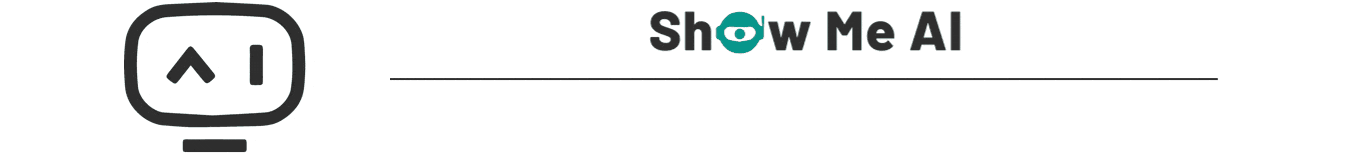# 斯坦福NLP课程 | 第11讲 – NLP中的卷积神经网络

10℃

## 🚀 优质资源分享 🚀

🧡 Python实战微信订餐小程序 🧡 进阶级 本课程是python flask+微信小程序的完美结合，从项目搭建到腾讯云部署上线，打造一个全栈订餐系统。
💛Python量化交易实战💛 入门级 手把手带你打造一个易扩展、更安全、效率更高的量化交易系统ShowMeAI斯坦福CS224n《自然语言处理与深度学习(Natural Language Processing with Deep Learning)》课程的全部课件，做了中文翻译和注释，并制作成了GIF动图！# 引言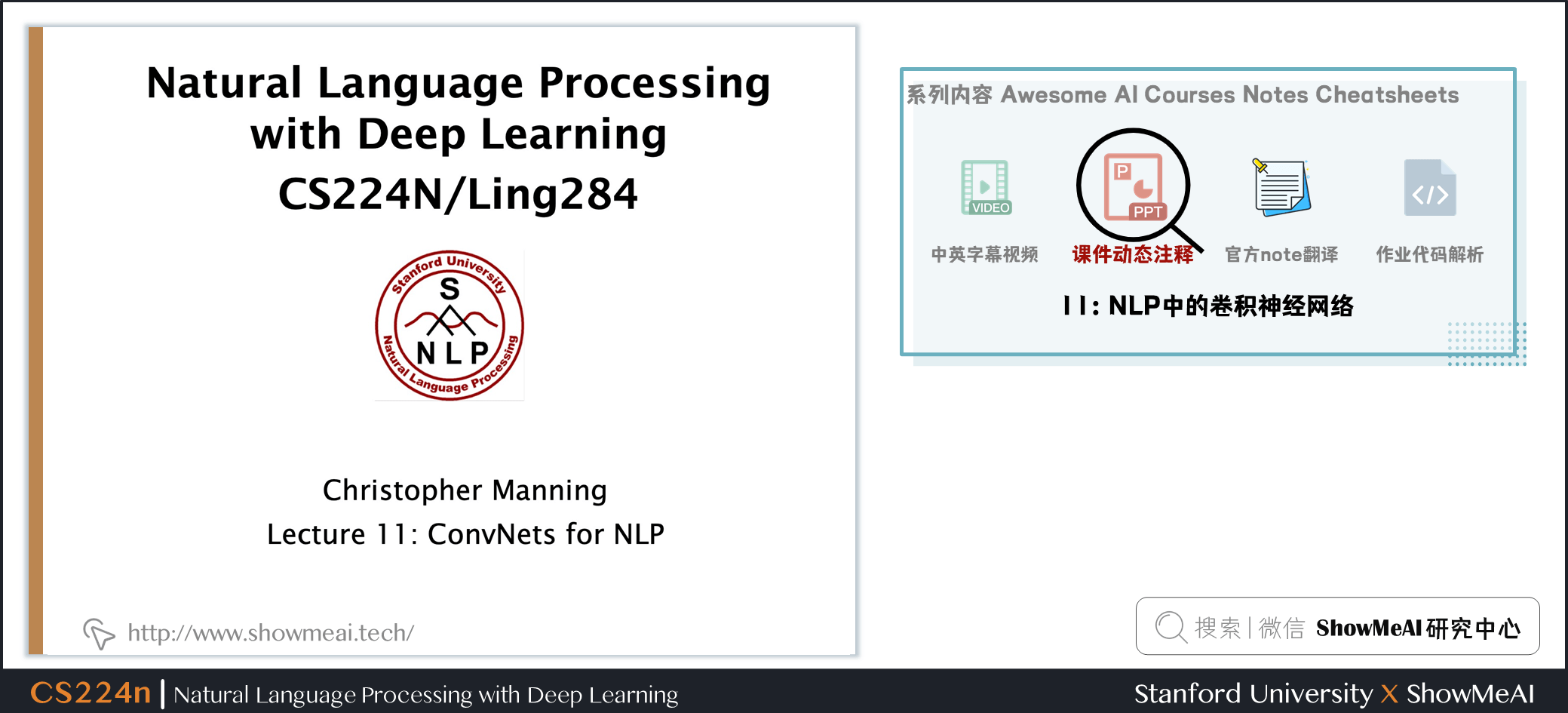## 授课计划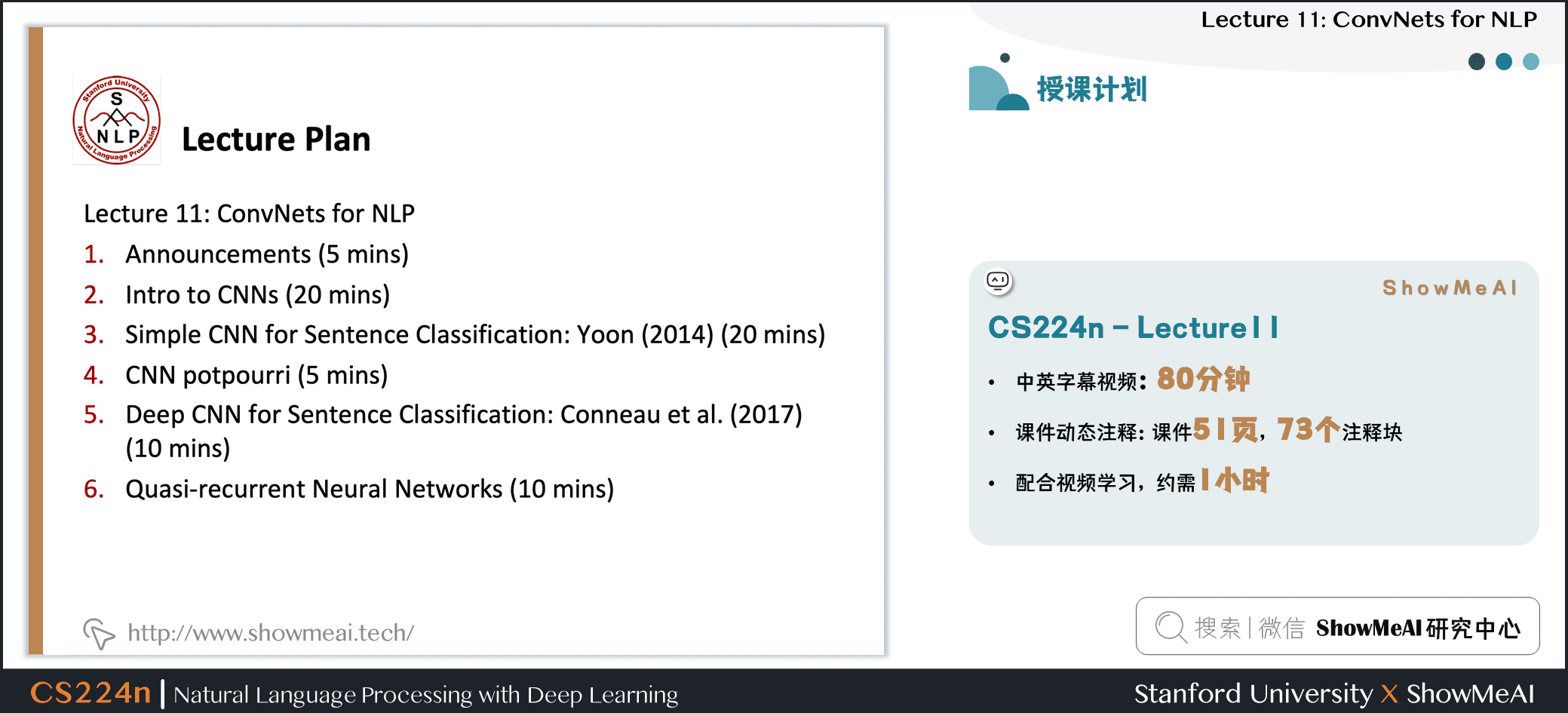• Announcements
• Intro to CNNs / 卷积神经网络介绍
• Simple CNN for Sentence Classification: Yoon (2014) / 应用CNN做文本分类
• CNN potpourri / CNN 细节
• Deep CNN for Sentence Classification: Conneauet al. (2017) / 深度CNN用于文本分类
• Quasi-recurrent Neural Networks / Q-RNN模型

## 欢迎来到课程的下半部分！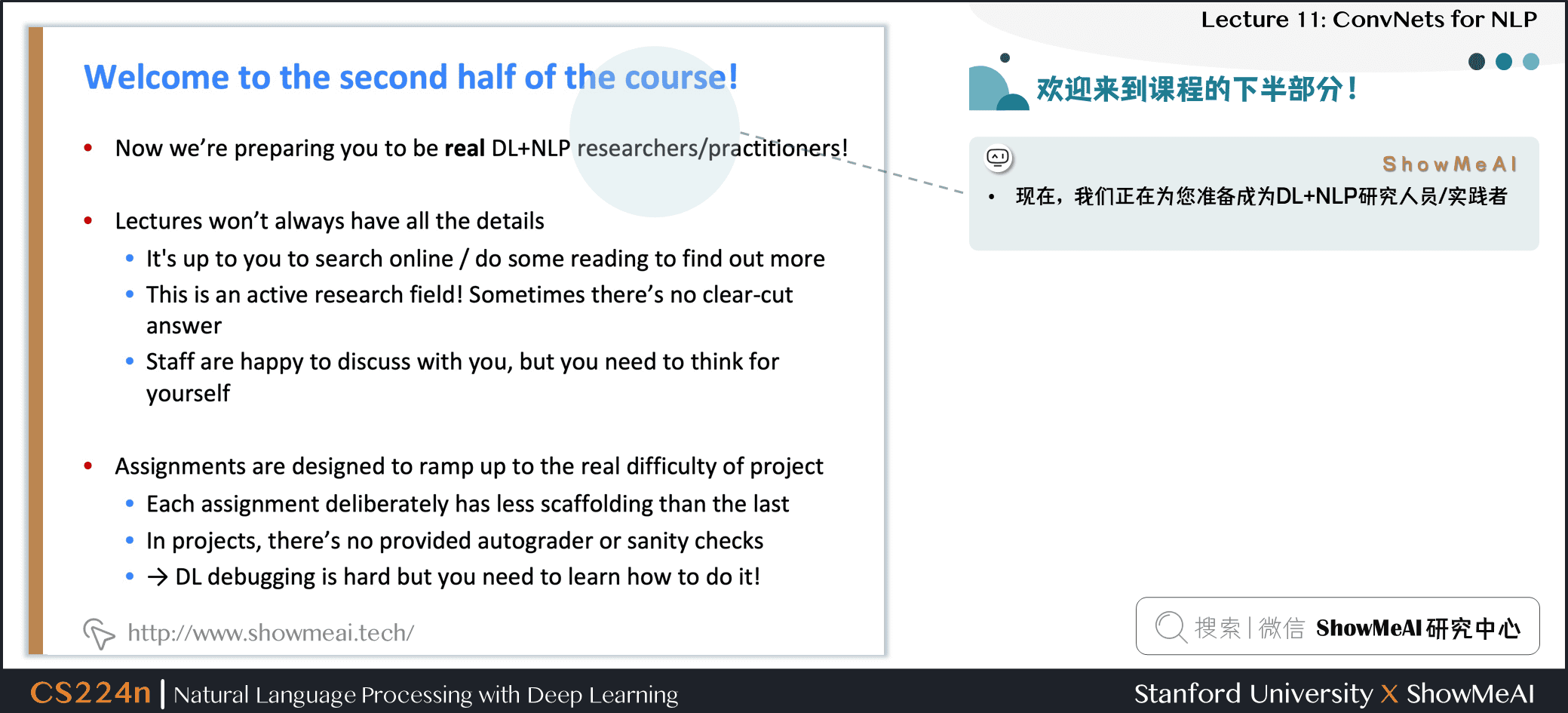• 现在，我们正在为你准备成为 DL+NLP 研究人员/实践者
• 课程不会总是有所有的细节

• 这取决于你在网上搜索/阅读来了解更多
• 这是一个活跃的研究领域，有时候没有明确的答案
• Staff 很乐意与你讨论，但你需要自己思考
• 作业的设计是为了应付项目的真正困难

• 每个任务都故意比上一个任务有更少的帮助材料
• DL 调试很困难，但是你需要学习如何进行调试！

## 书籍推荐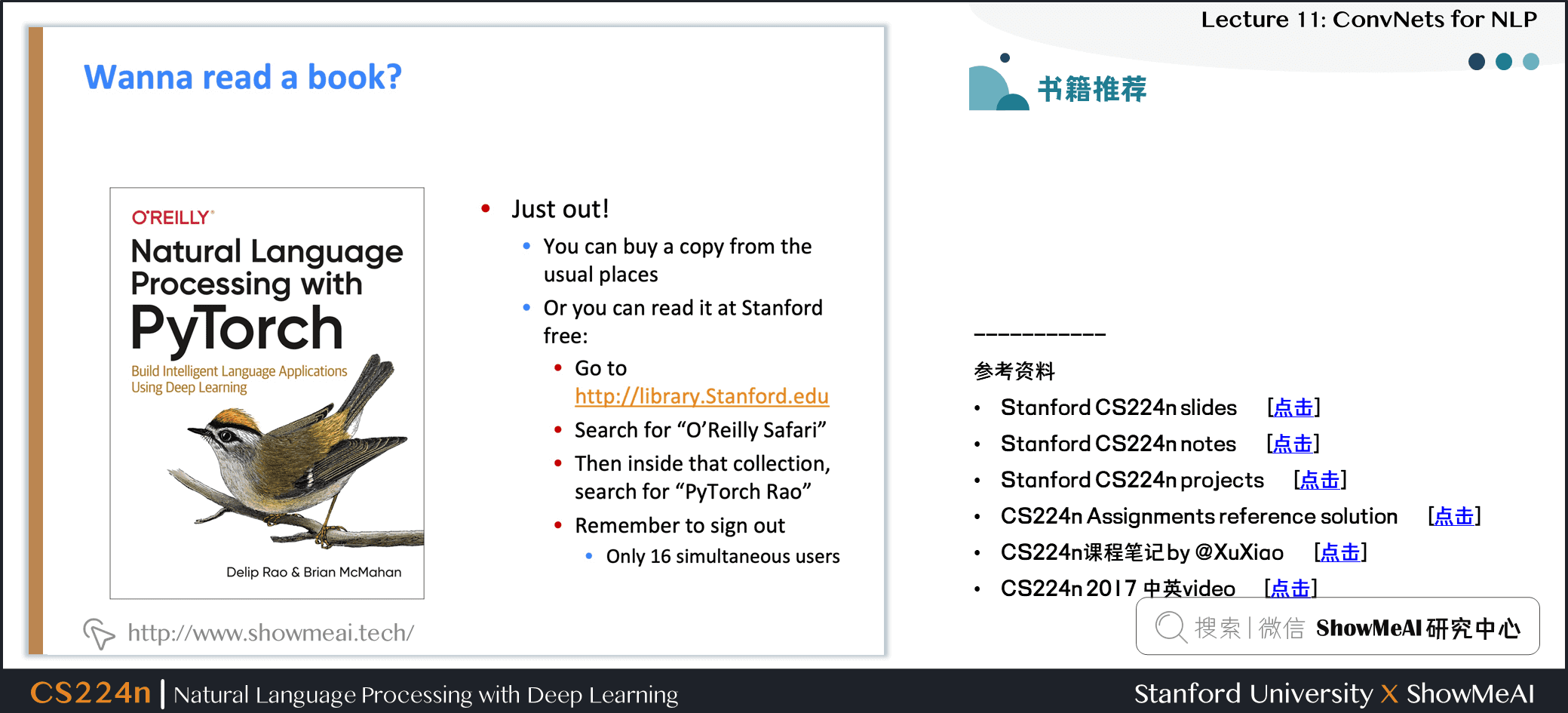Natural Language Processing with PyTorch: Build Intelligent Language Applications Using Deep Learning

• Delip Rao & Goku Mohandas

# 1.卷积神经网络介绍

(卷积神经网络相关内容也可以参考ShowMeAI的对吴恩达老师课程的总结文章 深度学习教程 | 卷积神经网络解读

## 1.1 从RNN到CNN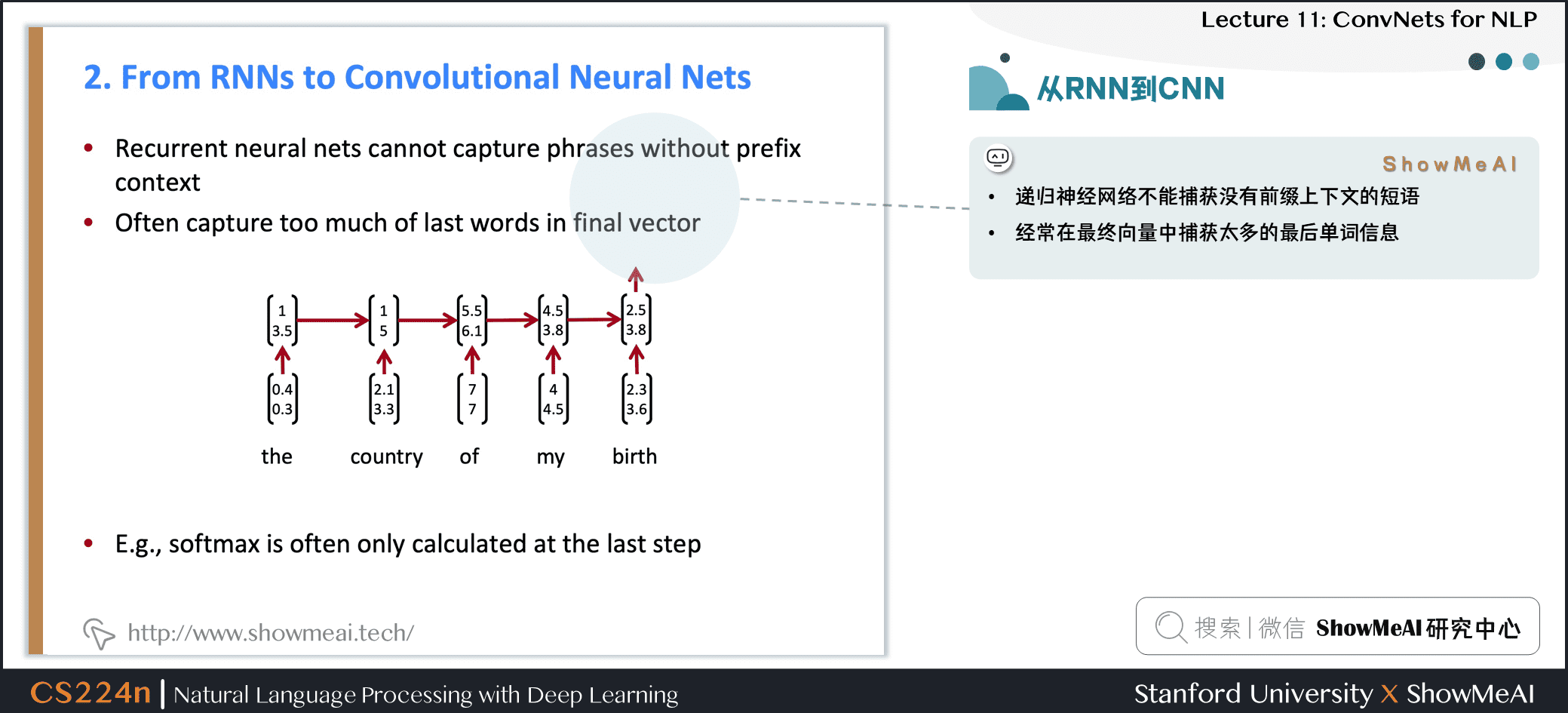• 循环神经网络不能捕获没有前缀上下文的短语

• 经常在最终向量中捕获的信息太多来自于最后的一些词汇内容

• 例如：softmax通常只在最后一步计算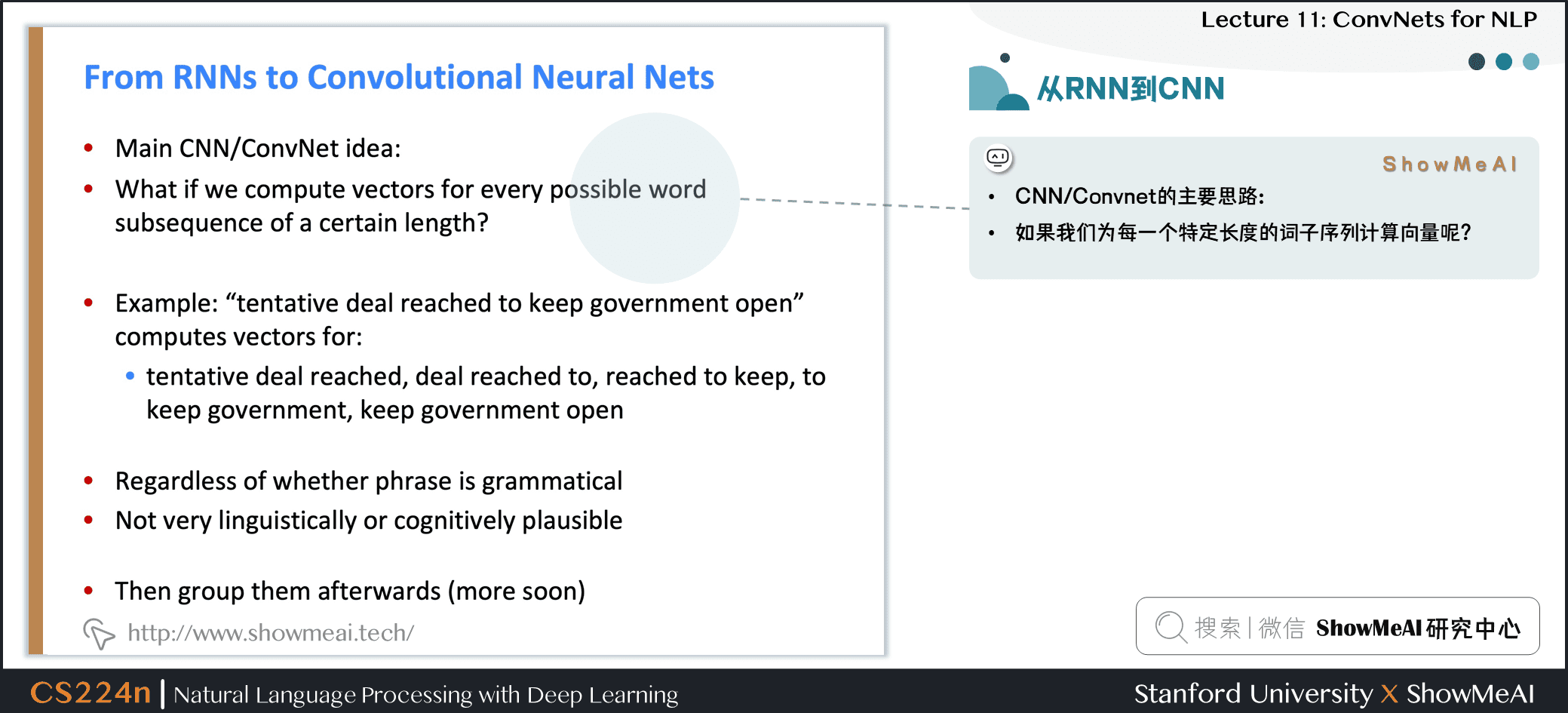• CNN / Convnet 的主要思路

• 如果我们为每一个特定长度的词子序列计算向量呢？
• 例如：tentative deal reached to keep government open

• 计算的向量为

• tentative deal reached, deal reached to, reached to keep, to keep government, keep government open
• 不管短语是否合乎语法

• 在语言学上或认知上不太可信

• 然后将它们分组 (很快)

## 1.2 CNN 卷积神经网络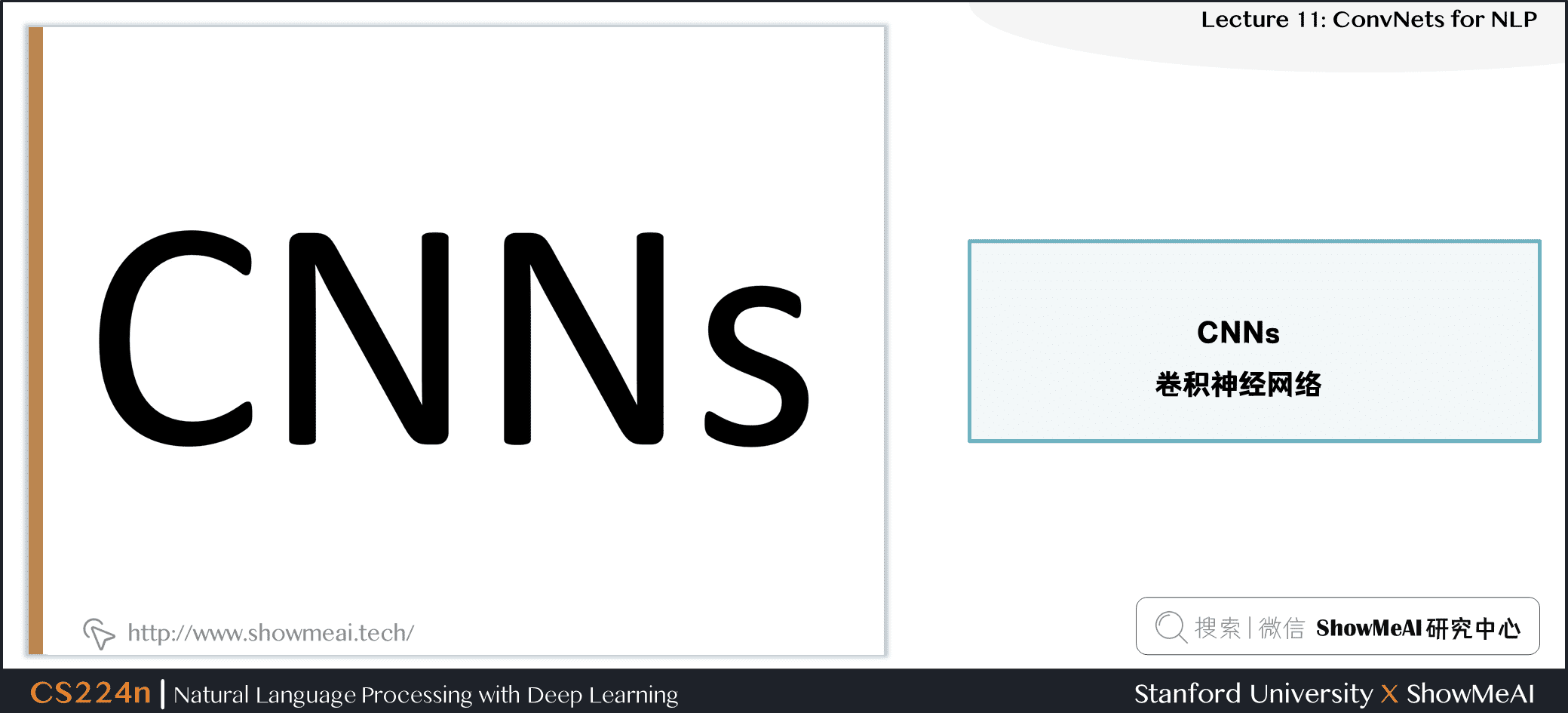## 1.3 什么是卷积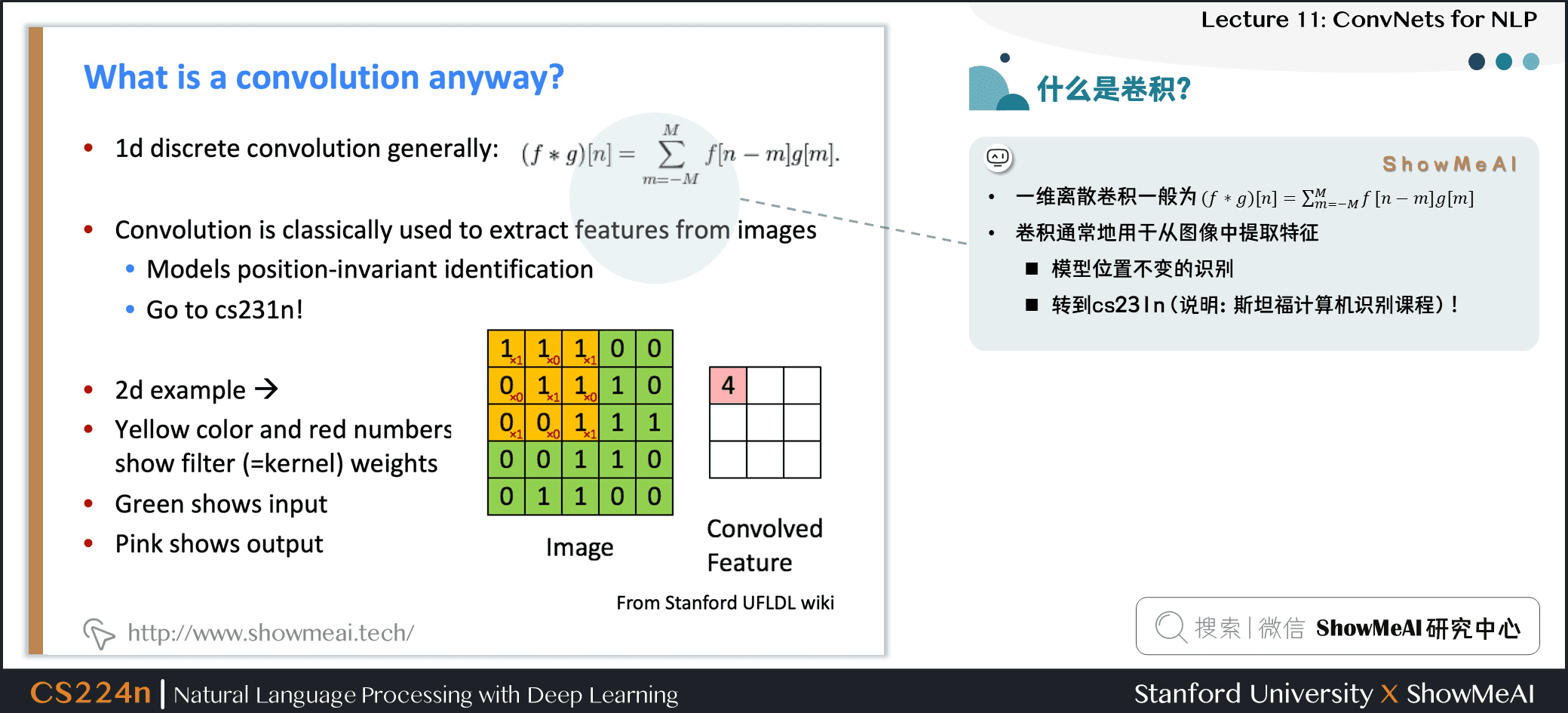• 一维离散卷积一般为：(f∗g)[n]=∑Mm=−Mf[n−m]gm[n]=∑m=−MMf[n−m]g[m](f \ast g)[n]=\sum_{m=-M}^{M} f[n-m] g[m]

• 卷积通常地用于从图像中提取特征

• 模型位置不变的识别
• 可以参考斯坦福深度学习与计算机视觉课程cs231n (也可以在ShowMeAI查阅 cs231n 系列笔记学习)
• 二维示例：

• 黄色和红色数字显示过滤器 (=内核) 权重
• 绿色显示输入
• 粉色显示输出

## 1.4 文本的一维卷积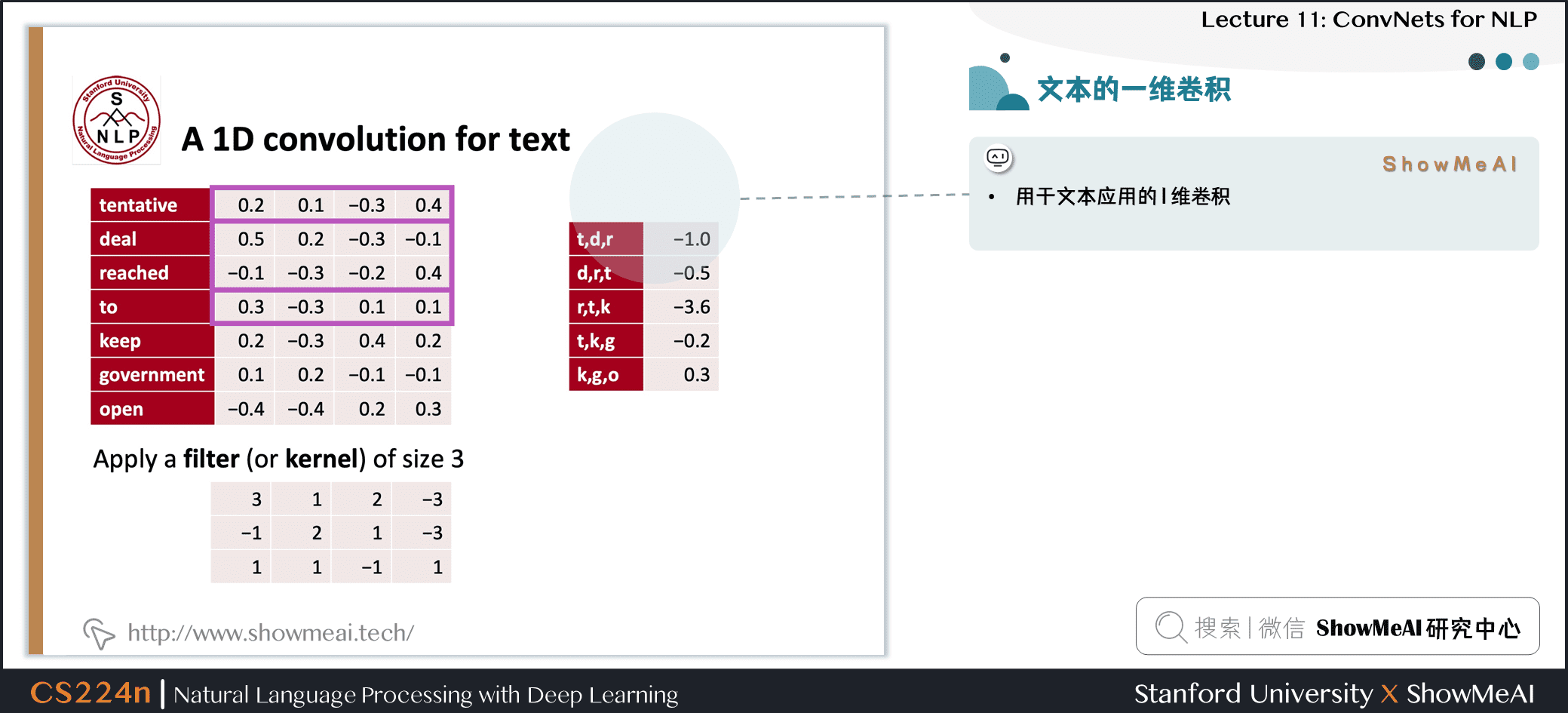• 用于文本应用的 1 维卷积

## 1.5 带填充的文本的一维卷积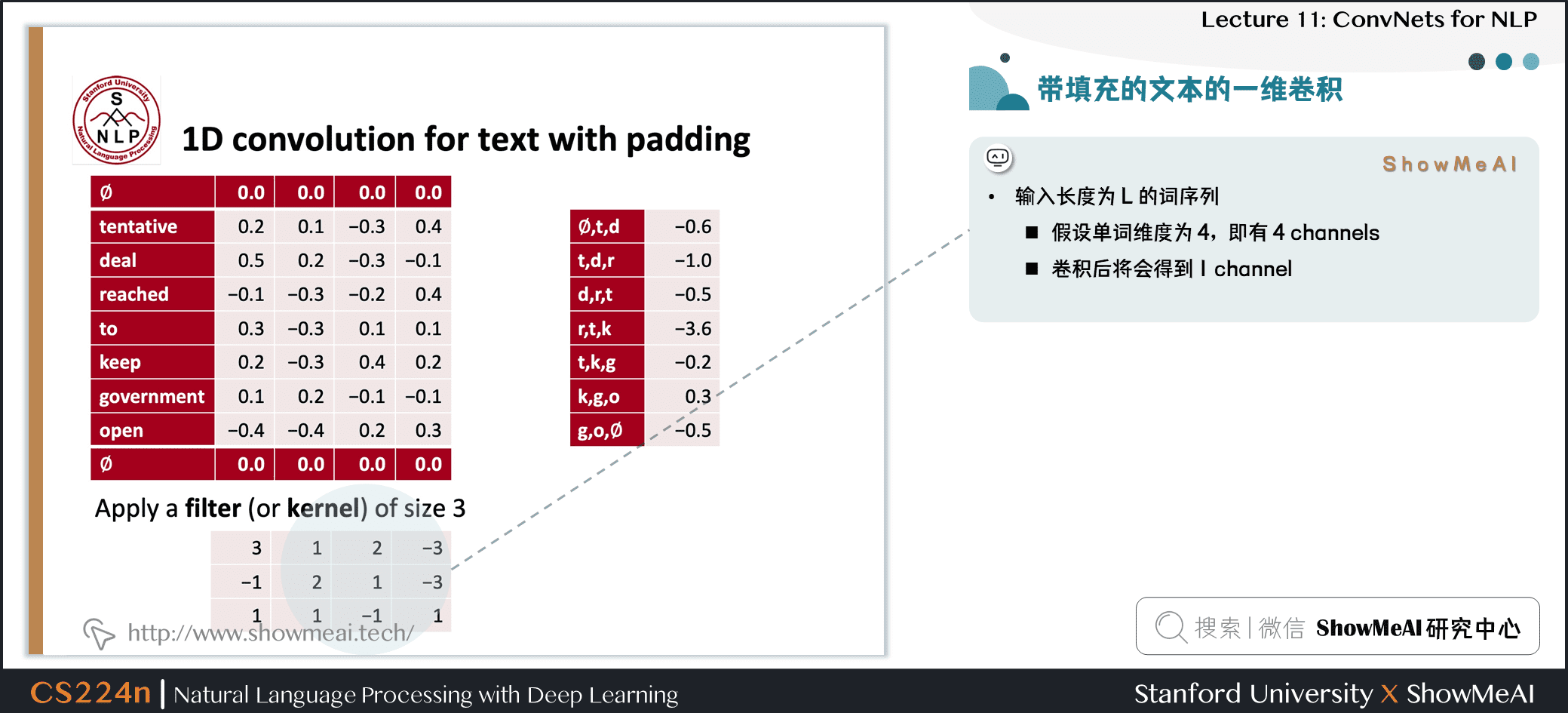• 输入长度为 LLL 的词序列

• 假设单词维度为 4，即有 4 channels
• 卷积后将会得到 1 channel
• 多个channel，则最终得到多个 channel 的输出，关注的文本潜在特征也不同

## 1.6 conv1d，随时间推移填充最大池化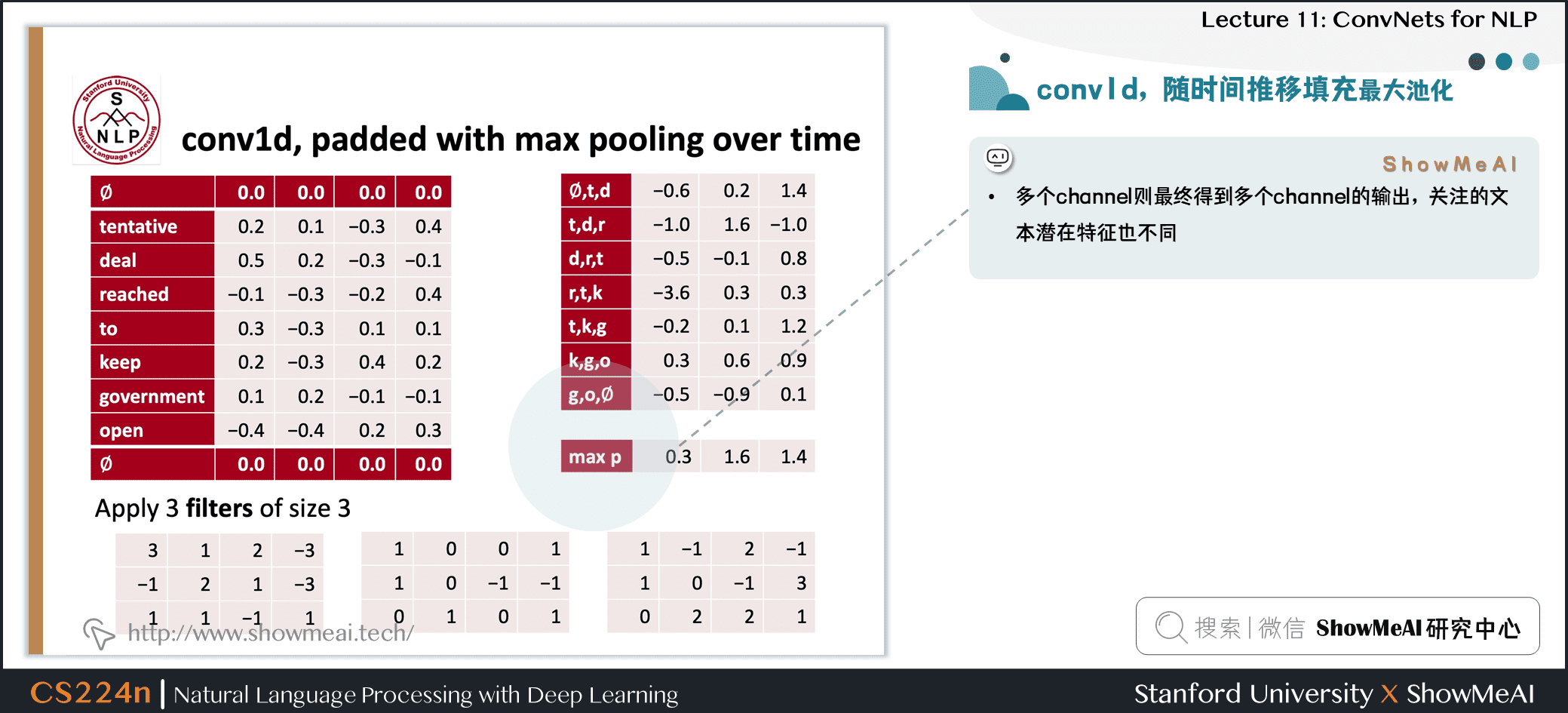• 平均池化对 feature map 求平均

## 1.7 PyTorch实现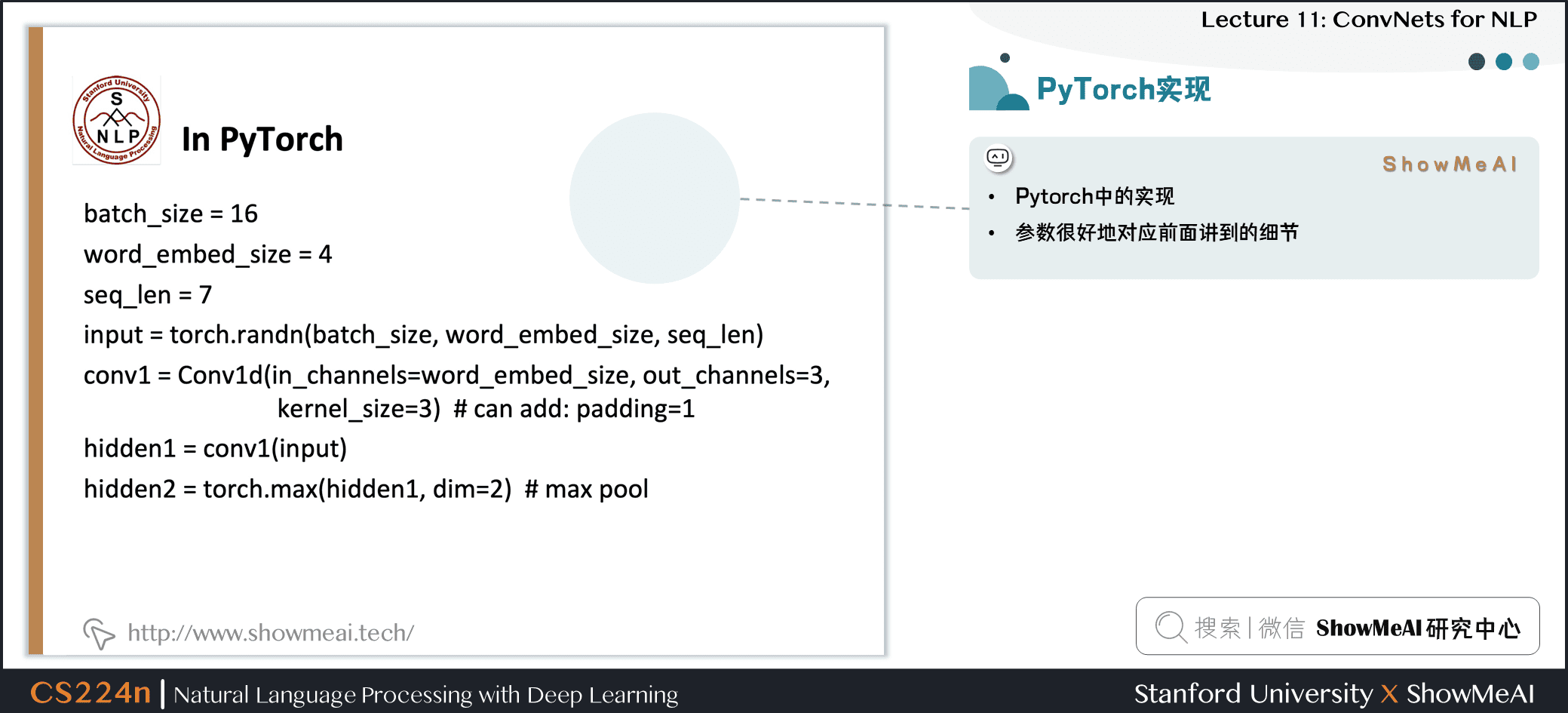• Pytorch中的实现：参数很好地对应前面讲到的细节

|  | batch\_size= 16 |
|  | word\_embed\_size= 4 |
|  | seq\_len= 7 |
|  | input = torch.randn(batch\_size, word\_embed\_size, seq\_len) |
|  | conv1 = Conv1d(in\_channels=word\_embed\_size, out\_channels=3, kernel\_size=3) # can add: padding=1  |
|  | hidden1 = conv1(input) |
|  | hidden2 = torch.max(hidden1, dim=2) # max pool |


## 1.8 步长 (这里为2)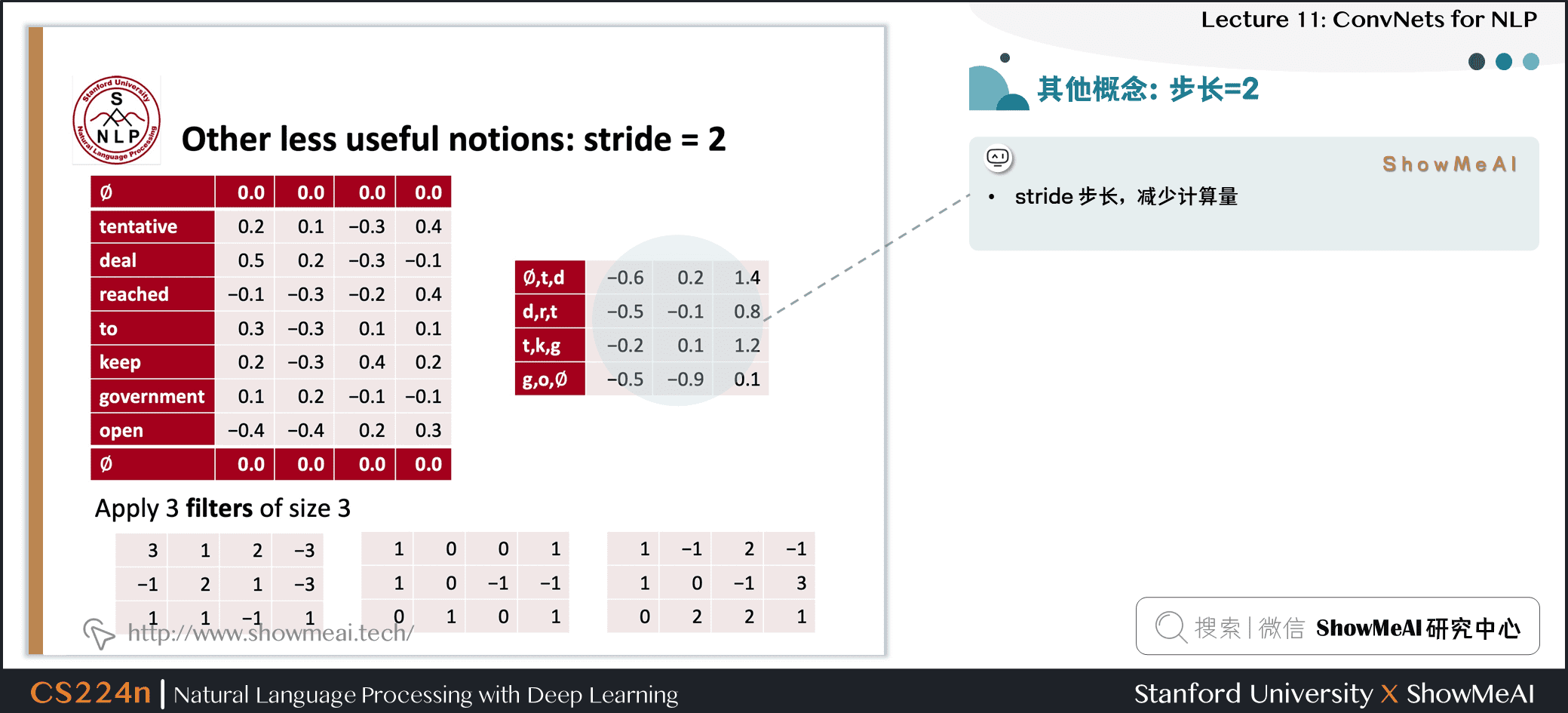• stride 步长，减少计算量

## 1.9 局部最大池化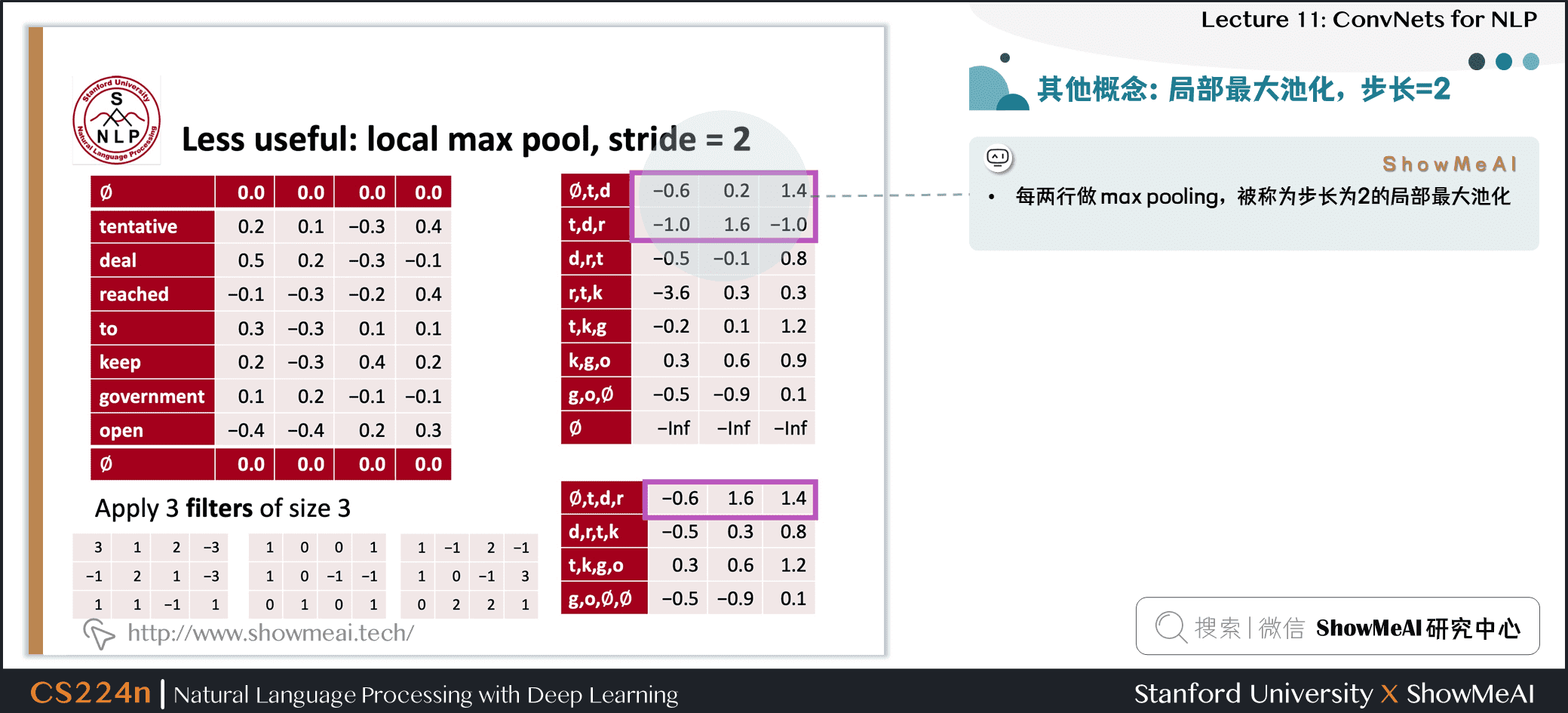• 每两行做 max pooling，被称为步长为 2 的局部最大池化

## 1.10 1维卷积的k-max pooling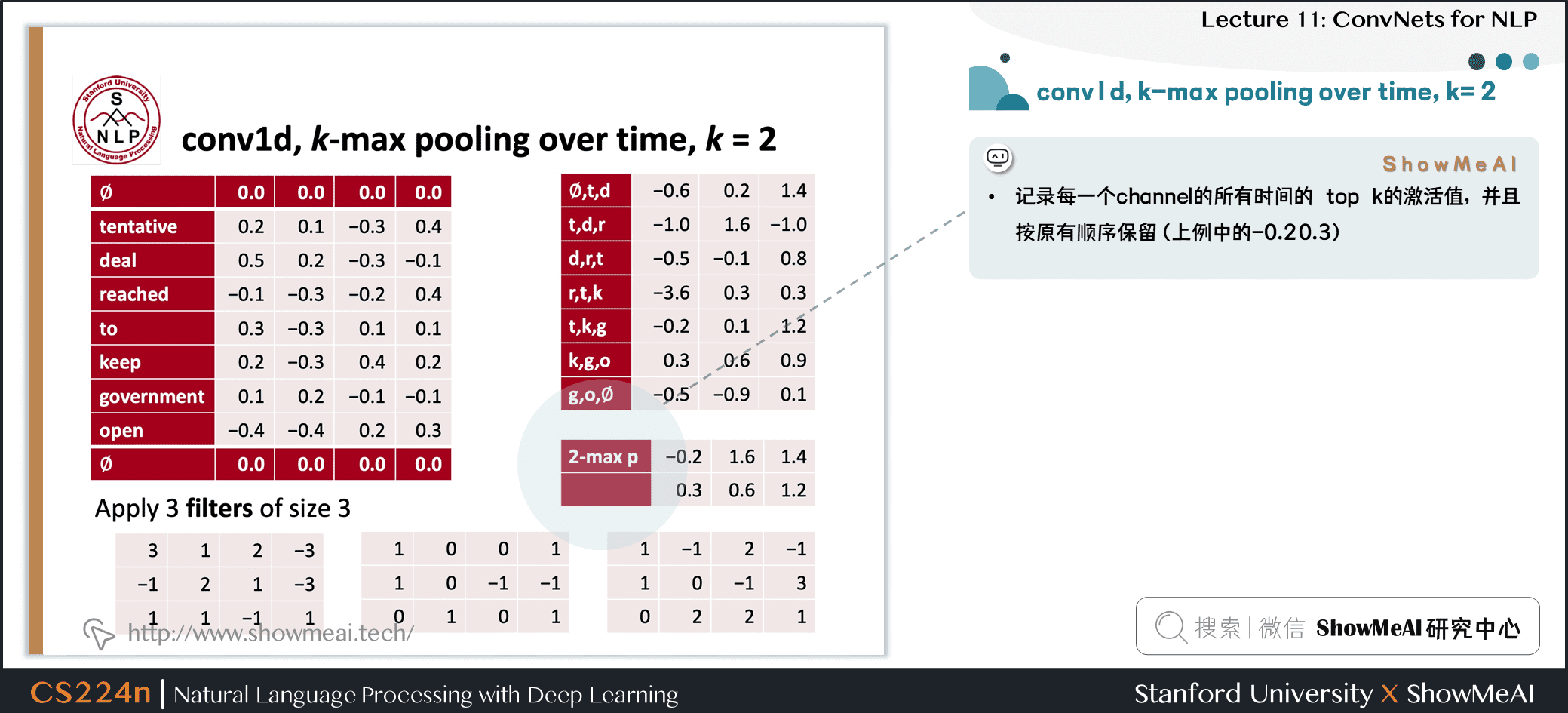• 记录每一个 channel 的所有时间的 top k 的激活值，并且按原有顺序保留(上例中的-0.2 0.3)

## 1.11 空洞卷积：dilation为2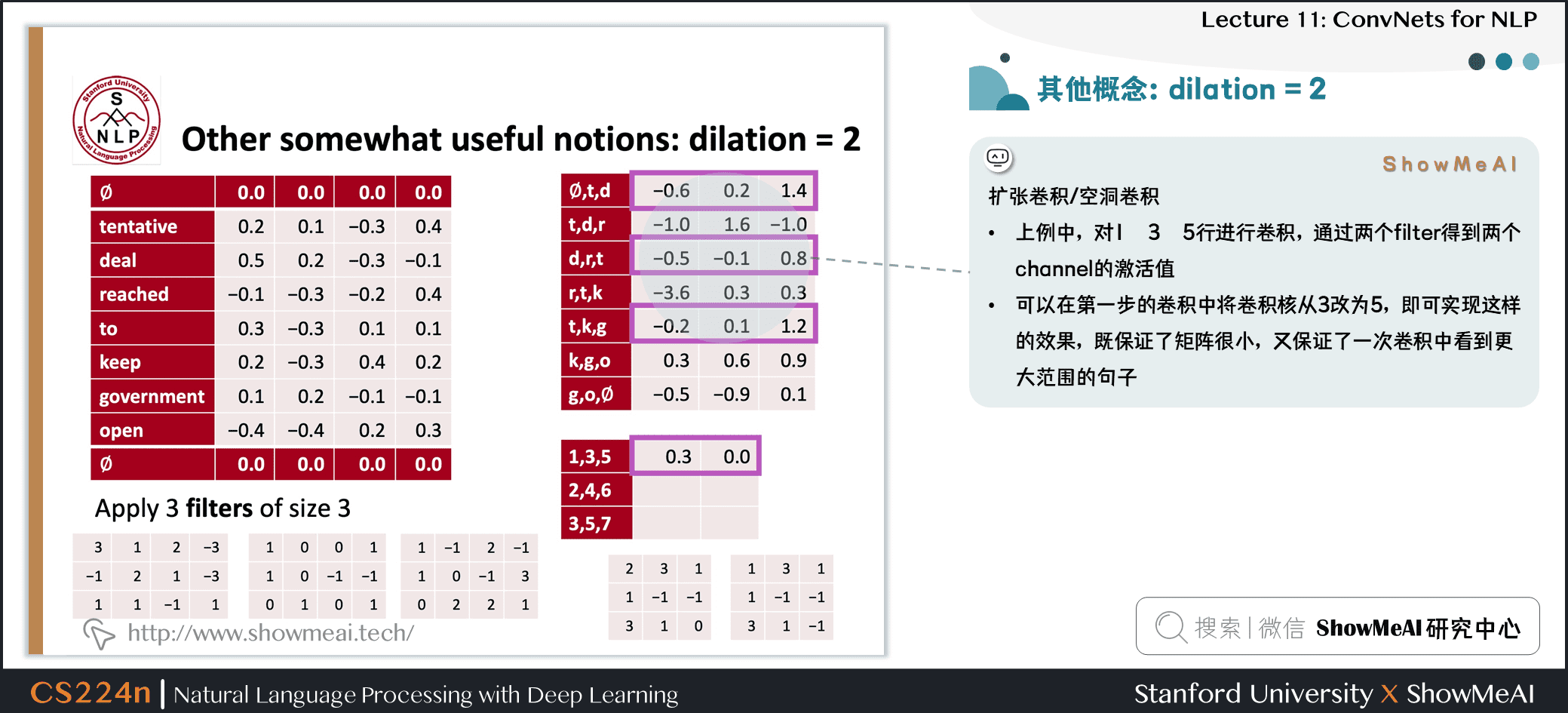• 上例中，对1 3 5行进行卷积，通过两个 filter 得到两个 channel 的激活值
• 可以在第一步的卷积中将卷积核从 3 改为 5，即可实现这样的效果，既保证了矩阵很小，又保证了一次卷积中看到更大范围的句子

• CNN中，一次能看一个句子的多少内容是很重要的概念
• 可以使用更大的 filter、扩张卷积或者增大卷积深度 (层数)

# 2.应用CNN做文本分类

## 2.1 用于句子分类的单层CNN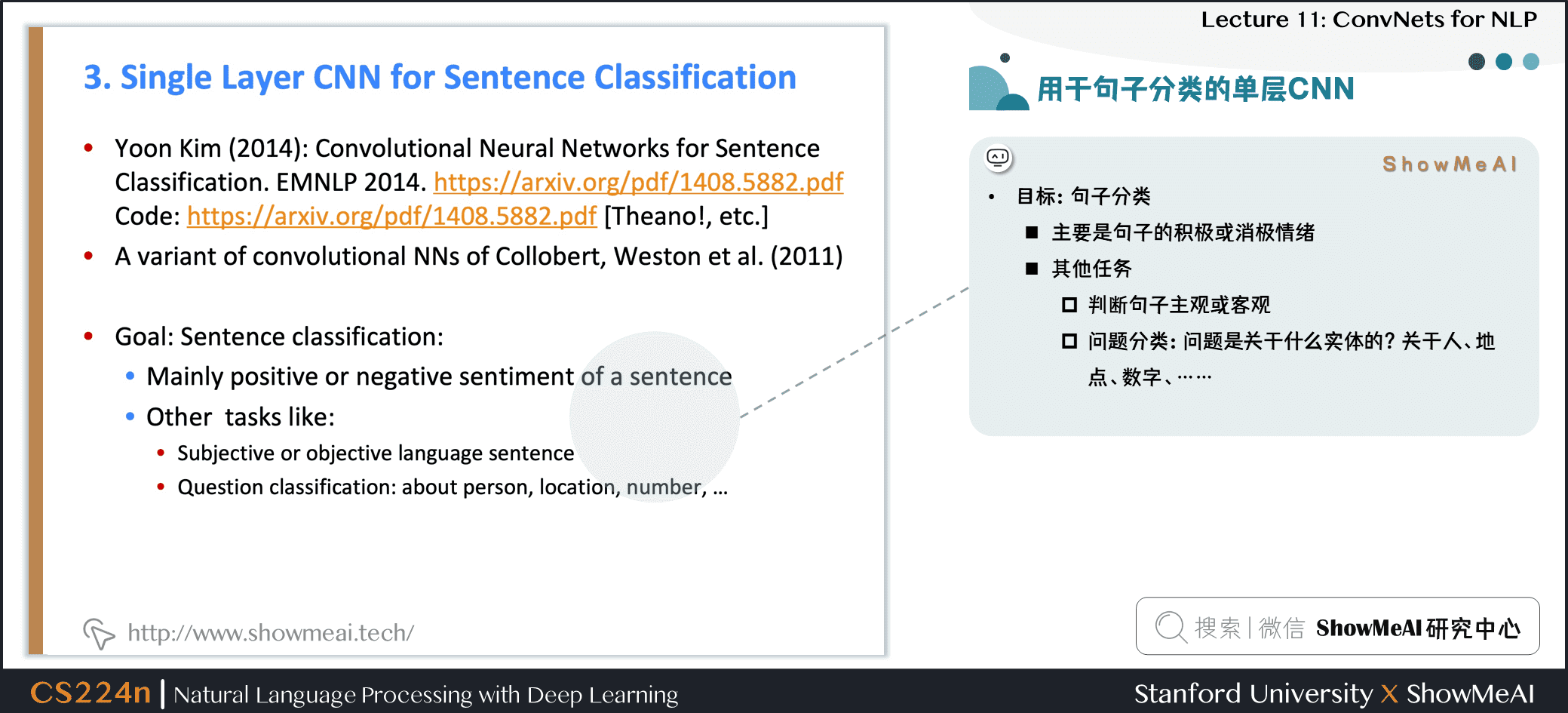• 目标：句子分类
• 主要是识别判断句子的积极或消极情绪
• 其他任务
• 判断句子主观或客观
• 问题分类：问题是关于什么实体的？关于人、地点、数字、……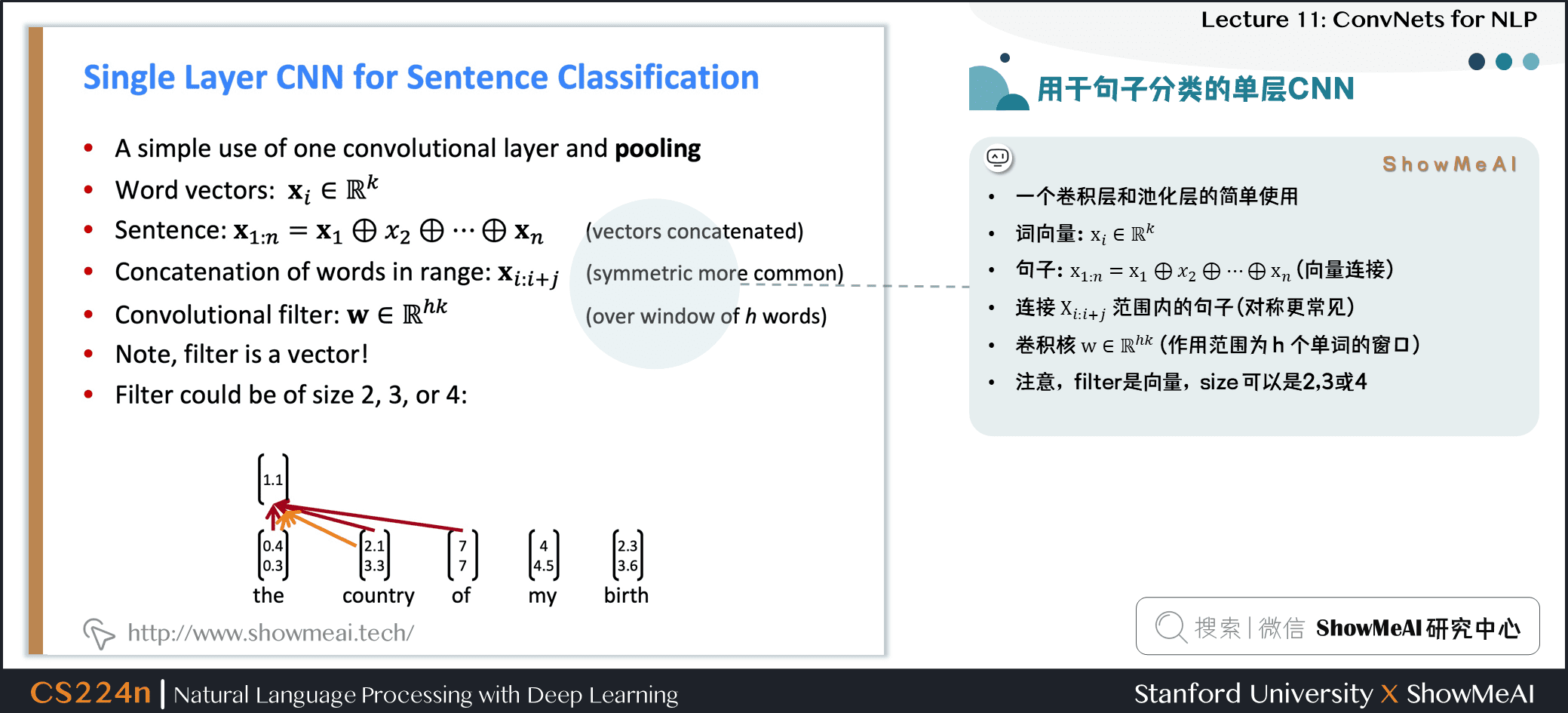• 一个卷积层和池化层的简单使用
• 词向量：xi∈Rkxi∈Rk\mathbf{x}_{i} \in \mathbb{R}^{k}
• 句子：x1:n=x1⊕x2⊕⋯⊕xnx1:n=x1⊕x2⊕⋯⊕xn\mathbf{x}_{1 : n}=\mathbf{x}_{1} \oplus x_{2} \oplus \cdots \oplus \mathbf{x}_{n} (向量连接)
• 连接 Xi:i+jXi:i+j\mathbf{X}_{i : i+j} 范围内的句子 (对称更常见)
• 卷积核 w∈Rhkw∈Rhk\mathbf{w} \in \mathbb{R}^{h k} (作用范围为 hhh 个单词的窗口)
• 注意，filter是向量，size 可以是2、3或4

## 2.2 单层CNN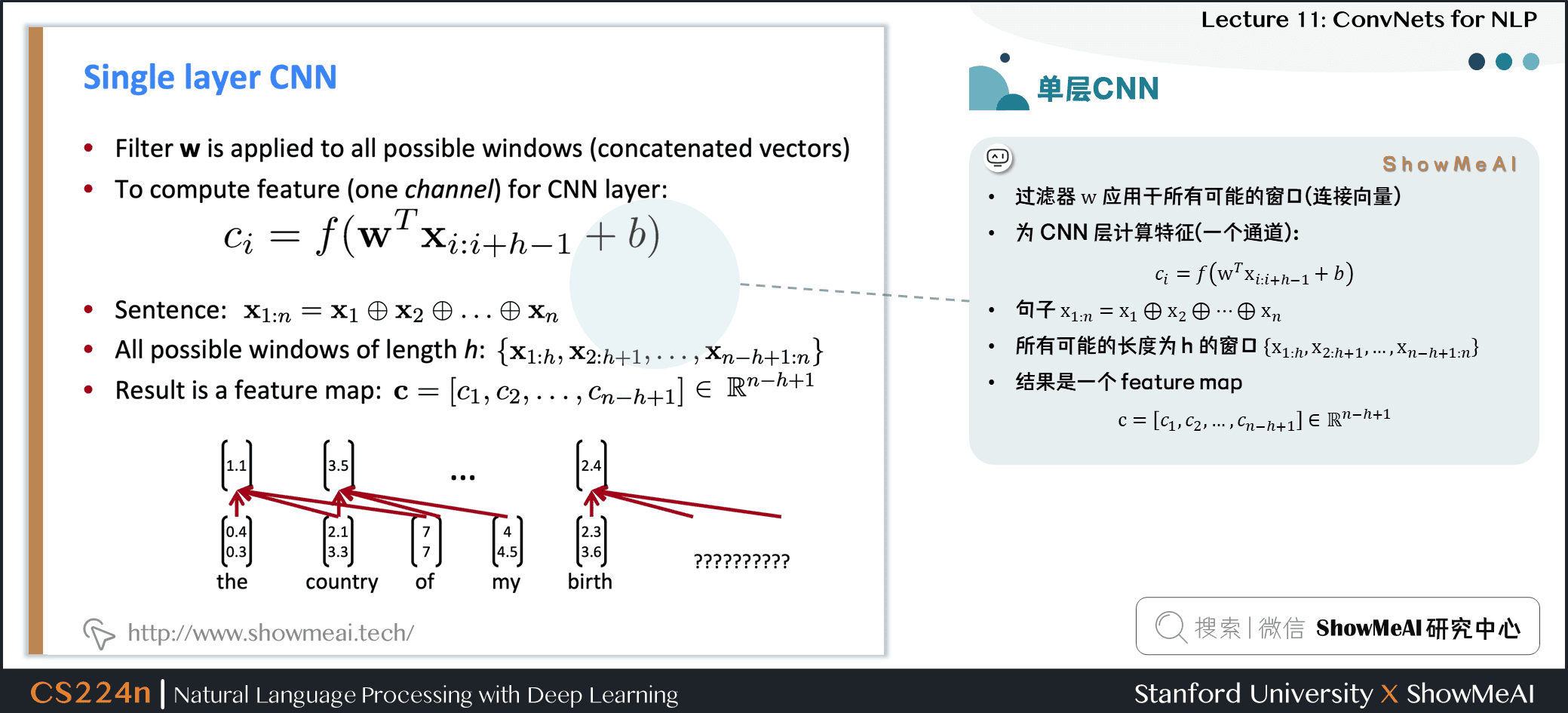• 过滤器 www 应用于所有可能的窗口(连接向量)
• 为CNN层计算特征(一个通道)

ci=f(wTxi:i+h−1+b)ci=f(wTxi:i+h−1+b)c_{i}=f\left(\mathbf{w}^{T} \mathbf{x}_{i : i+h-1}+b\right)

• 句子 x1:n=x1⊕x2⊕…⊕xnx1:n=x1⊕x2⊕…⊕xn\mathbf{x}_{1 : n}=\mathbf{x}_{1} \oplus \mathbf{x}_{2} \oplus \ldots \oplus \mathbf{x}_{n}
• 所有可能的长度为 hhh 的窗口 {x1:h,x2:h+1,…,xn−h+1:n}{x1:h,x2:h+1,…,xn−h+1:n}\left{\mathbf{x}_{1 : h}, \mathbf{x}_{2 : h+1}, \dots, \mathbf{x}_{n-h+1 : n}\right}
• 结果是一个 feature map c=[c1,c2,…,cn−h+1]∈Rn−h+1c=[c1,c2,…,cn−h+1]∈Rn−h+1\mathbf{c}=\left[c_{1}, c_{2}, \dots, c_{n-h+1}\right] \in \mathbb{R}^{n-h+1}

## 2.3 池化与通道数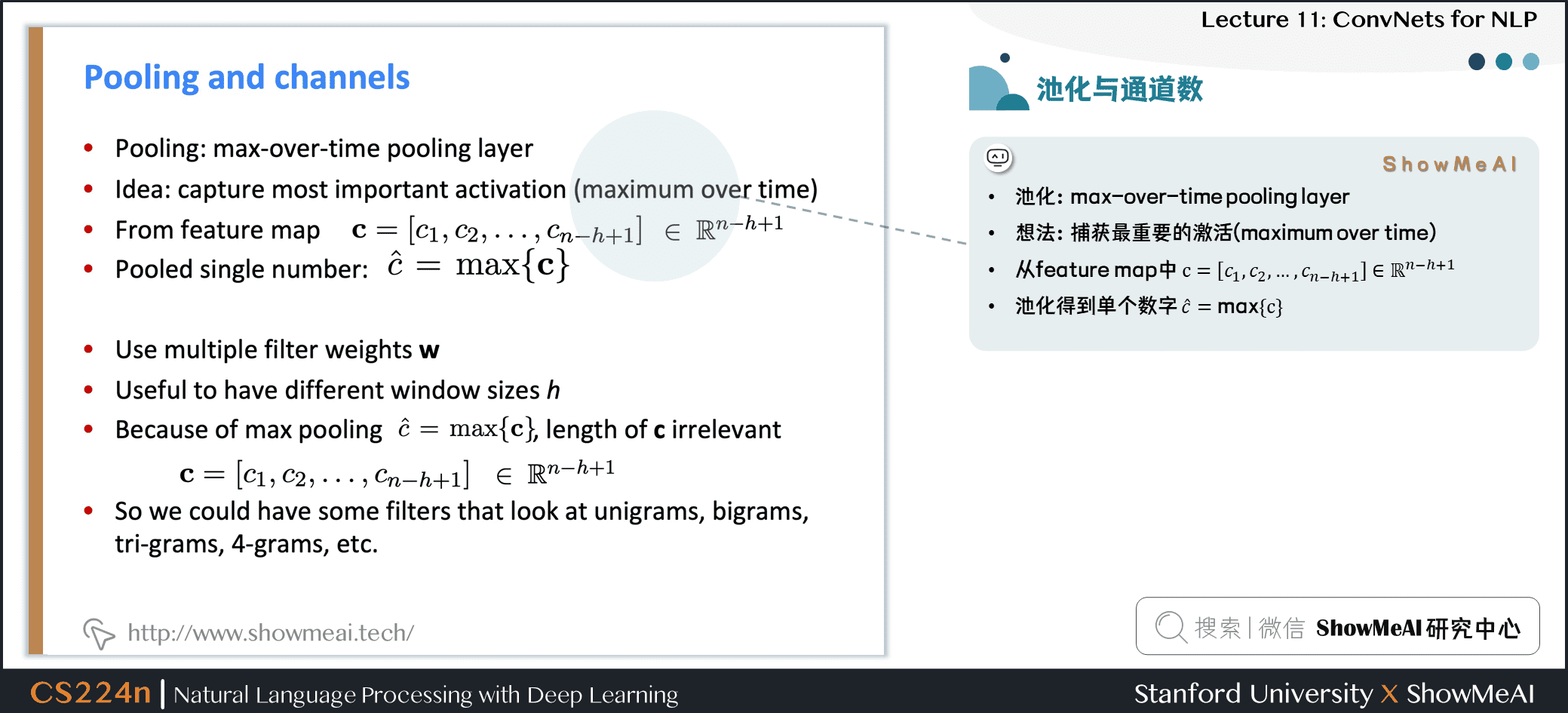• 池化：max-over-time pooling layer

• 想法：捕获最重要的激活(maximum over time)

• 从feature map中 c=[c1,c2,…,cn−h+1]∈Rn−h+1c=[c1,c2,…,cn−h+1]∈Rn−h+1\mathbf{c}=\left[c_{1}, c_{2}, \dots, c_{n-h+1}\right] \in \mathbb{R}^{n-h+1}

• 池化得到单个数字 c^=max{c}c^=max{c}\hat{c}=\max {\mathbf{c}}

• 使用多个过滤器权重 www

• 不同窗口大小 hhh 是有用的

• 由于最大池化 c^=max{c}c^=max{c}\hat{c}=\max {\mathbf{c}}，和 ccc 的长度无关

c=[c1,c2,…,cn−h+1]∈Rn−h+1c=[c1,c2,…,cn−h+1]∈Rn−h+1\mathbf{c}=\left[c_{1}, c_{2}, \dots, c_{n-h+1}\right] \in \mathbb{R}^{n-h+1}

• 所以我们可以有一些 filters 来观察 unigrams、bigrams、tri-grams、4-grams等等

## 2.4 多通道输入数据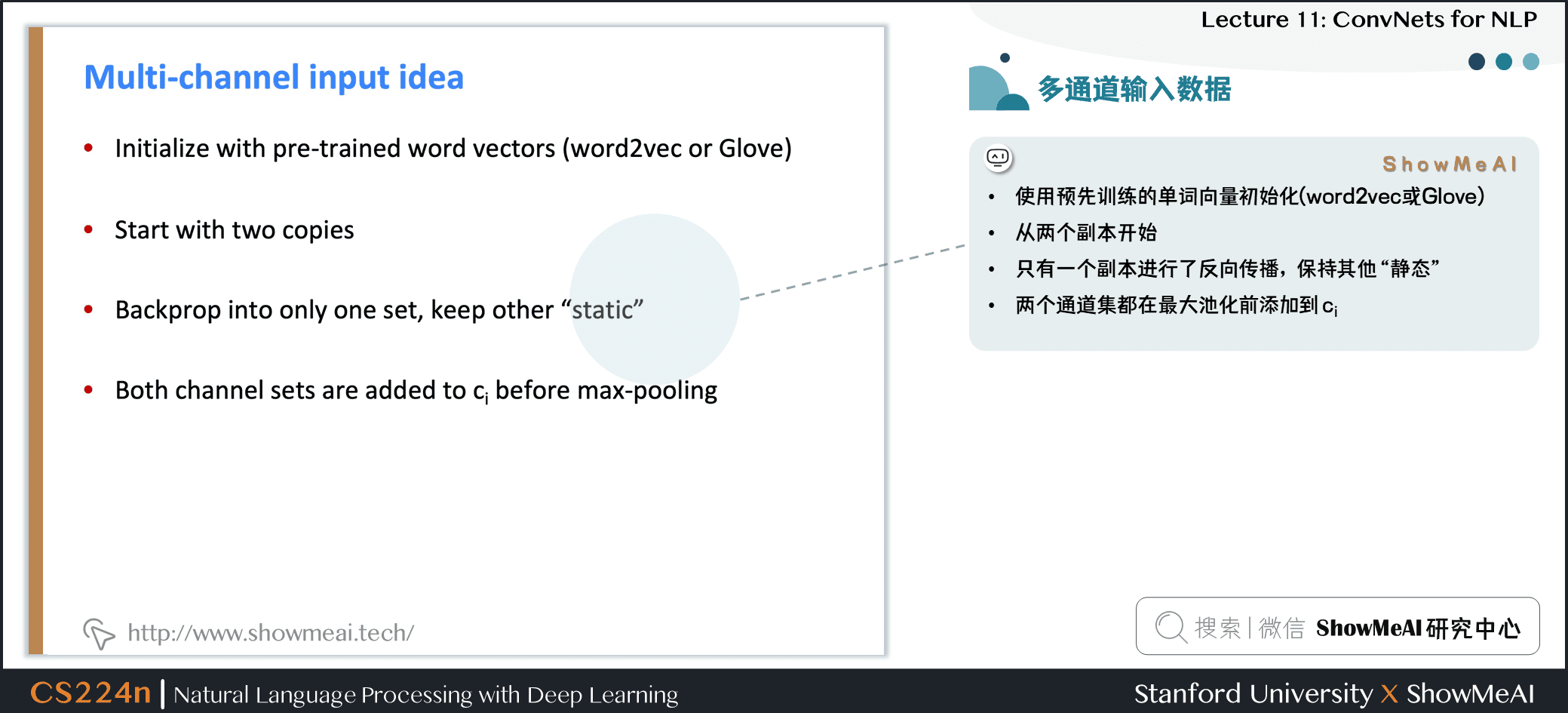• 使用预先训练的单词向量初始化 (word2vec 或 Glove)
• 从两个副本开始
• 只对1个副本进行了反向传播，其他保持静态
• 两个通道集都在最大池化前添加到 cicic_i

## 2.5 Classification after one CNN layer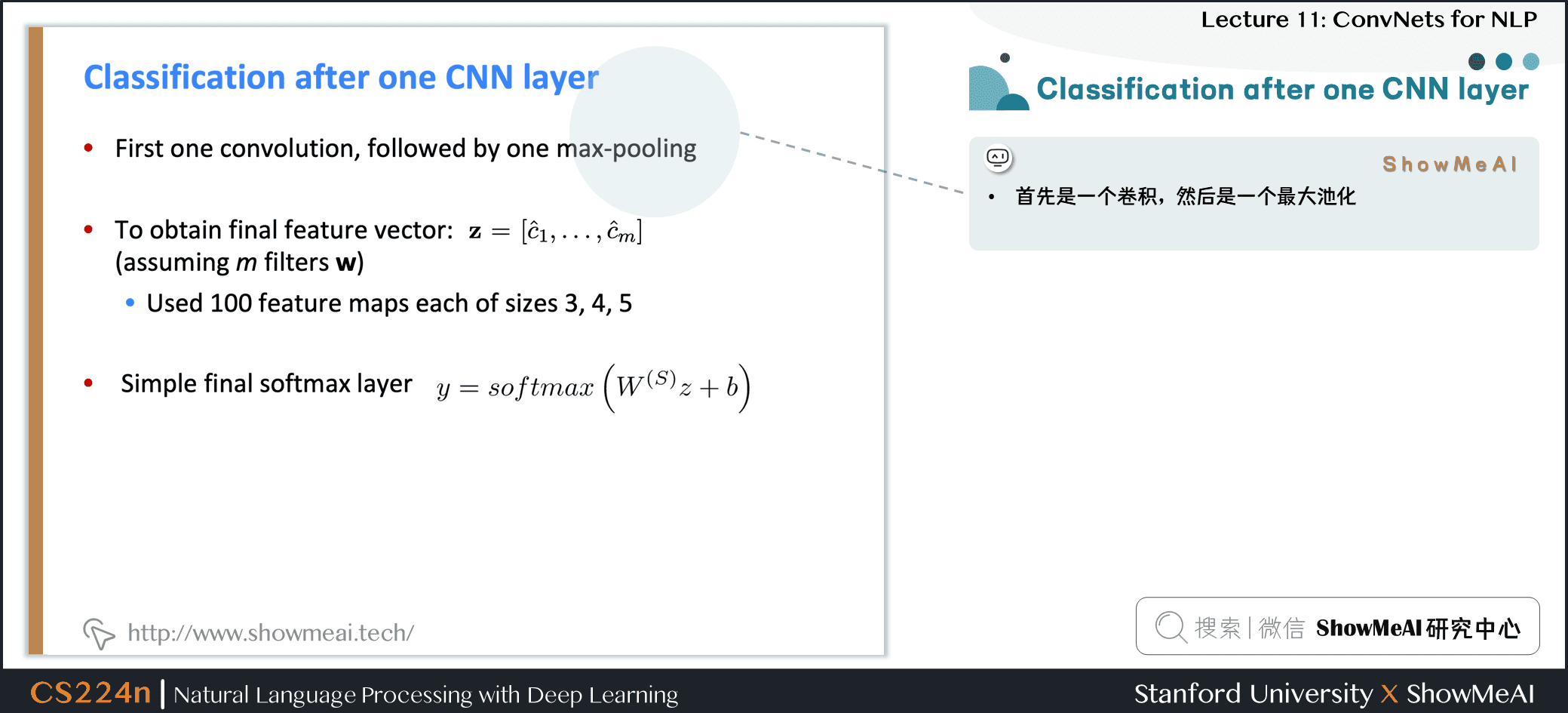• 首先是一个卷积，然后是一个最大池化

• 为了获得最终的特征向量 z=[c^1,…,c^m]z=[c^1,…,c^m]\mathbf{z}=\left[\hat{c}_{1}, \dots, \hat{c}_{m}\right]

• 假设我们有 mmm 个卷积核 (滤波器filter) www
• 使用100个大小分别为3、4、5的特征图
• 最终是简单的 softmax layer y=softmax(W(S)z+b)y=softmax⁡(W(S)z+b)y=\operatorname{softmax}\left(W^{(S)} z+b\right)

• https://arxiv.org/pdf/1510.03820.pdf
• 输入长度为 7 的一句话，每个词的维度是 5 ，即输入矩阵是 7×57×57 \times 5
• 使用不同的 filter_size : (2,3,4)，并且每个 size 都是用两个 filter，获得两个 channel 的 feature，即共计 6 个 filter
• 对每个 filter 的 feature 进行 1-max pooling 后，拼接得到 6 维的向量，并使用 softmax 后再获得二分类结果

## 2.6 Regularization 正则化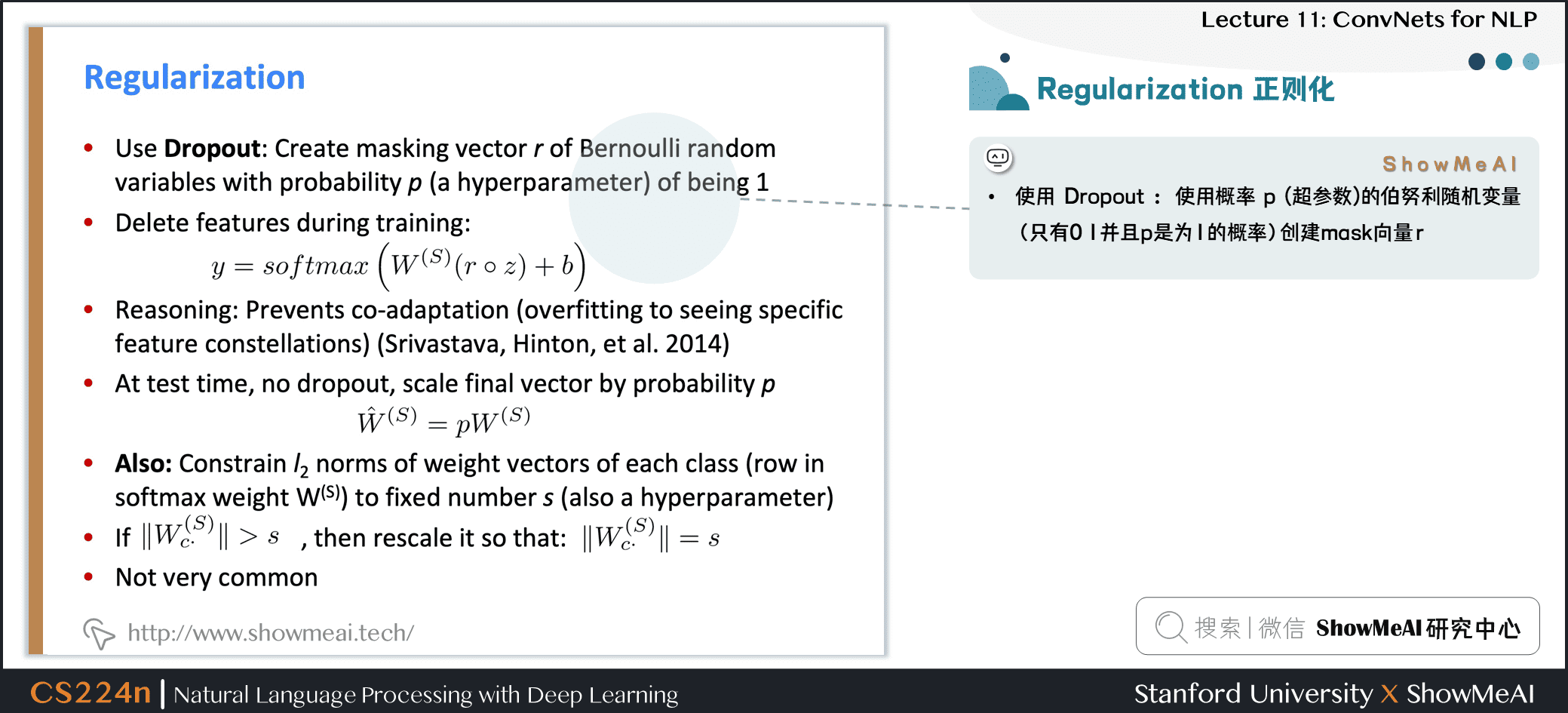• 使用 Dropout：使用概率 ppp (超参数) 的伯努利随机变量(只有0 1并且 ppp 是为 111 的概率)创建 mask 向量 rrr

• 训练过程中删除特征

y=softmax(W(S)(r∘z)+b)y=softmax⁡(W(S)(r∘z)+b)y=\operatorname{softmax}\left(W^{(S)}(r \circ z)+b\right)

• 解释：防止互相适应(对特定特征的过度拟合)
• 在测试时不适用 Dropout，使用概率 ppp 缩放最终向量

W^(S)=pW(S)W^(S)=pW(S)\hat{W}^{(S)}=p W^{(S)}

• 此外：限制每个类的权重向量的 L2 Norm (softmax 权重 W(S)W(S)W^{(S)} 的每一行) 不超过固定数 sss (也是超参数)
• 如果 ∥∥W(S)c∥∥>s‖Wc(S)‖>s\left|W_{c}^{(S)}\right|>s ，则重新缩放为 ∥∥W(S)c∥∥=s‖Wc(S)‖=s\left|W_{c}^{(S)}\right|=s
• 不是很常见

# 3.CNN细节

## 3.1 CNN参数讨论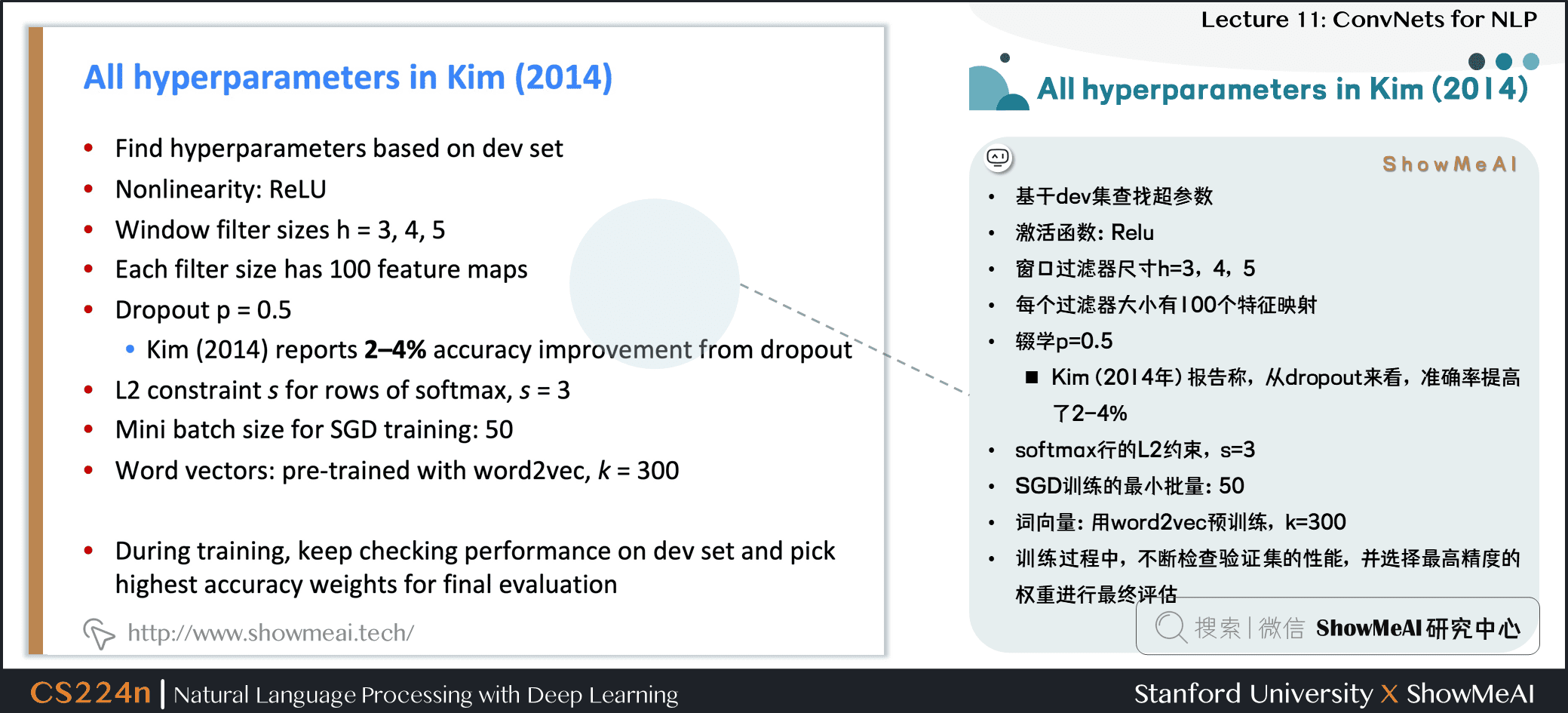• 基于验证集 (dev) 调整超参数
• 激活函数：Relu
• 窗口过滤器尺寸h=3，4，5h=3，4，5h=3，4，5
• 每个过滤器大小有 100 个特征映射
• Dropoutp=0.5p=0.5p=0.5
• Kim(2014年) 报告称，从 Dropout 来看，准确率提高了 2−4%2−4%2 - 4 \%
• softmax行的 L2 约束，s=3s=3s=3
• SGD训练的最小批量：505050
• 词向量：用 word2vec 预训练，k=300k=300k=300
• 训练过程中，不断检查验证集的性能，并选择最高精度的权重进行最终评估

## 3.2 实验结果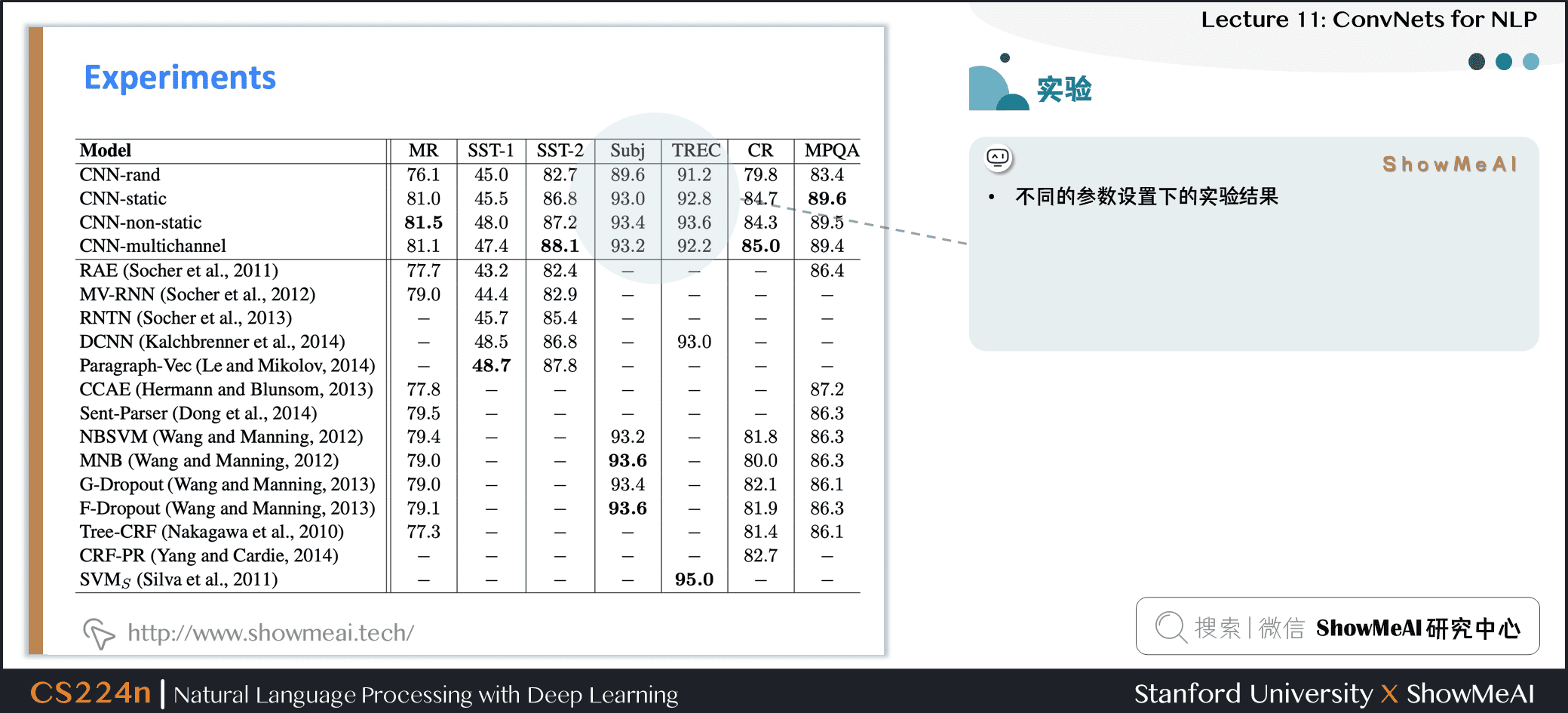• 不同的参数设置下的实验结果

## 3.3 对比CNN与RNN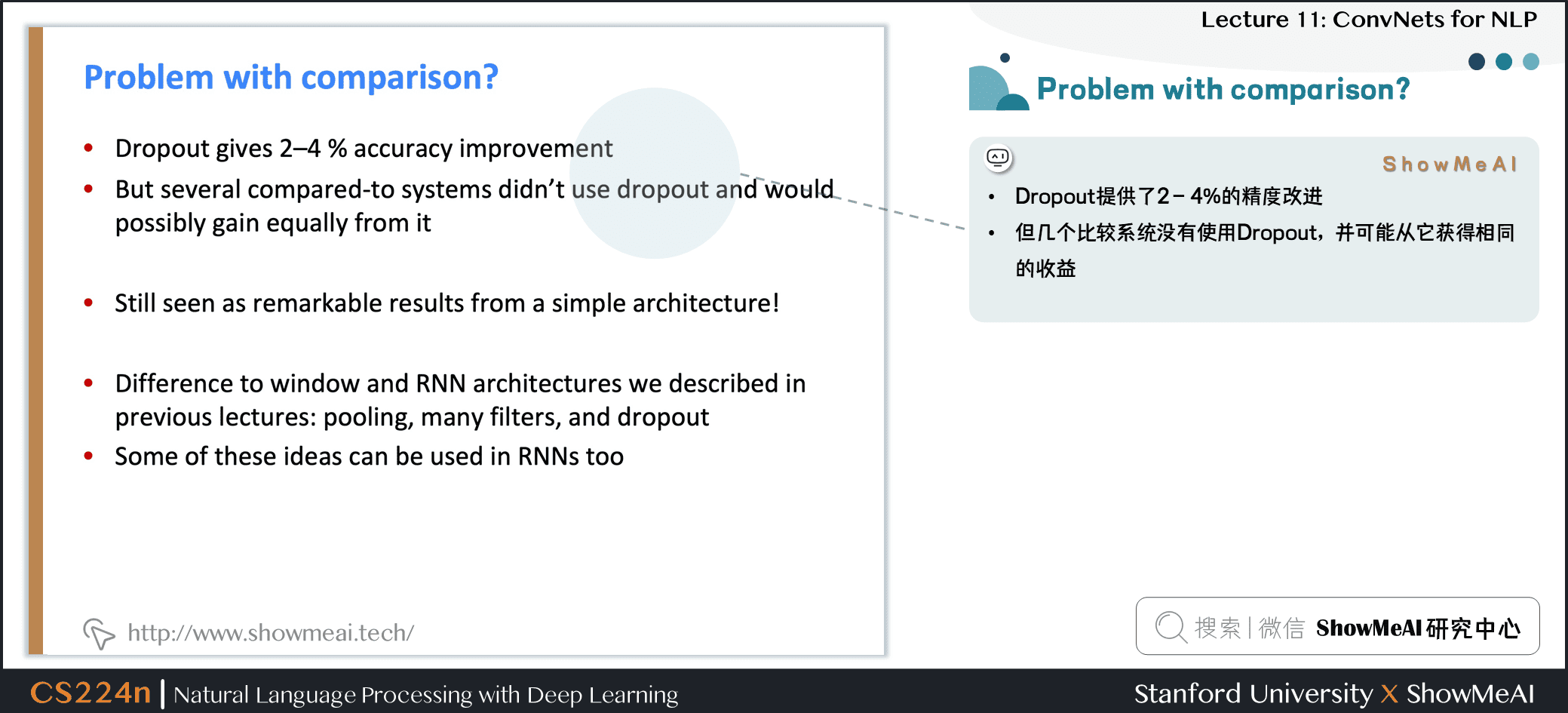• Dropout 提供了 2−4%2−4%2 - 4 \% 的精度改进

• 但几个比较系统没有使用 Dropout，并可能从它获得相同的收益

• 仍然被视为一个简单架构的显著结果

• 与我们在前几节课中描述的窗口和 RNN 架构的不同之处：池化、许多过滤器和 Dropout

• 这些想法中有的可以被用在 RNNs 中

## 3.4 模型对比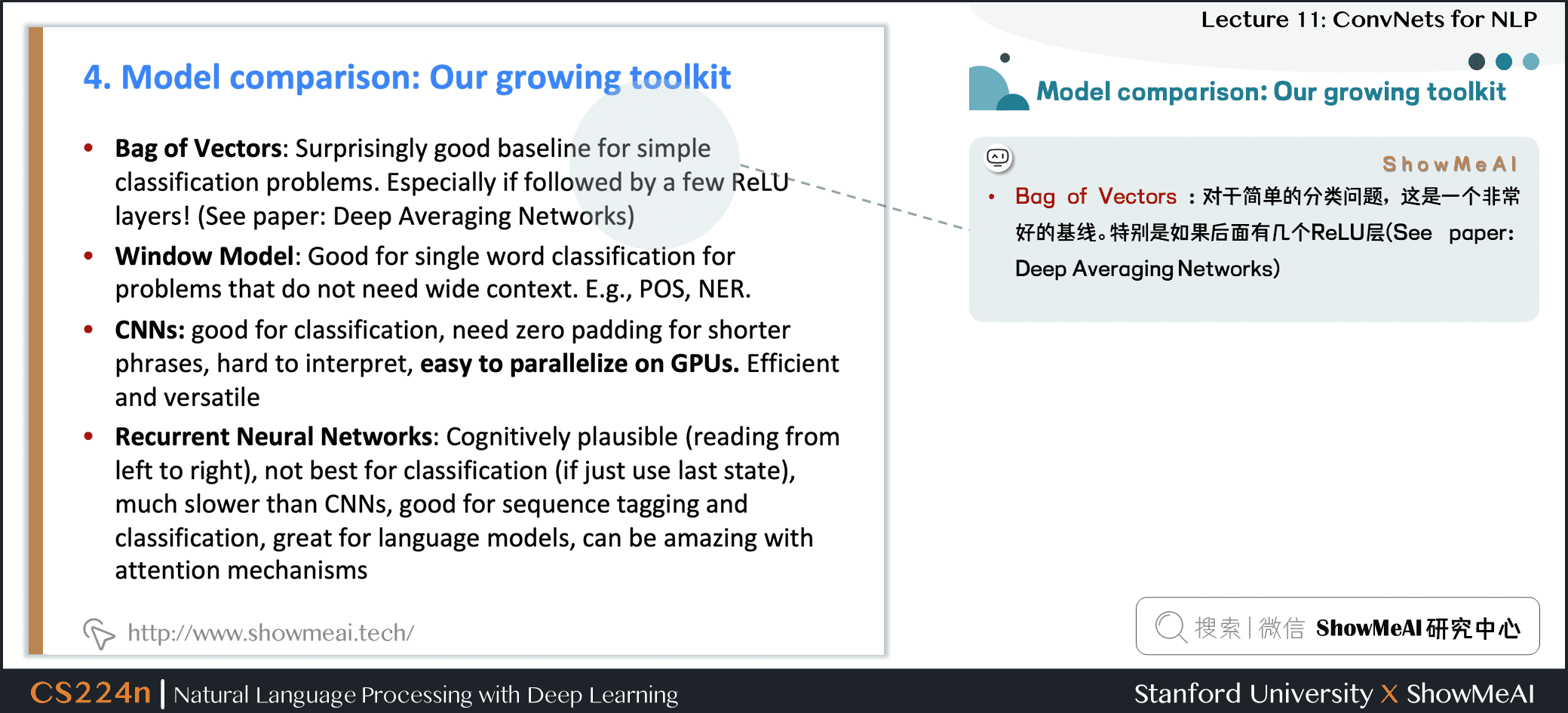• 词袋模型 / Bag of Vectors：对于简单的分类问题，这是一个非常好的基线。特别是如果后面有几个 ReLU 层 (See paper: Deep Averaging Networks)

• 词窗分类 / Window Model：对于不需要广泛上下文的问题 (即适用于 local 问题)，适合单字分类。例如 POS、NER

• 卷积神经网络 / CNN：适合分类，较短的短语需要零填充，难以解释，易于在 gpu 上并行化

• 循环神经网络 / RNN：从左到右的认知更加具有可信度，不适合分类 (如果只使用最后一种状态)，比 CNNs 慢得多，适合序列标记和分类以及语言模型，结合注意力机制时非常棒

• RNN对序列标记和分类之类的事情有很好的效果，以及语言模型预测下一个单词，并且结合注意力机制会取得很好的效果，但是对于某个句子的整体解释，CNN做的是更好的

## 3.5 跳接结构应用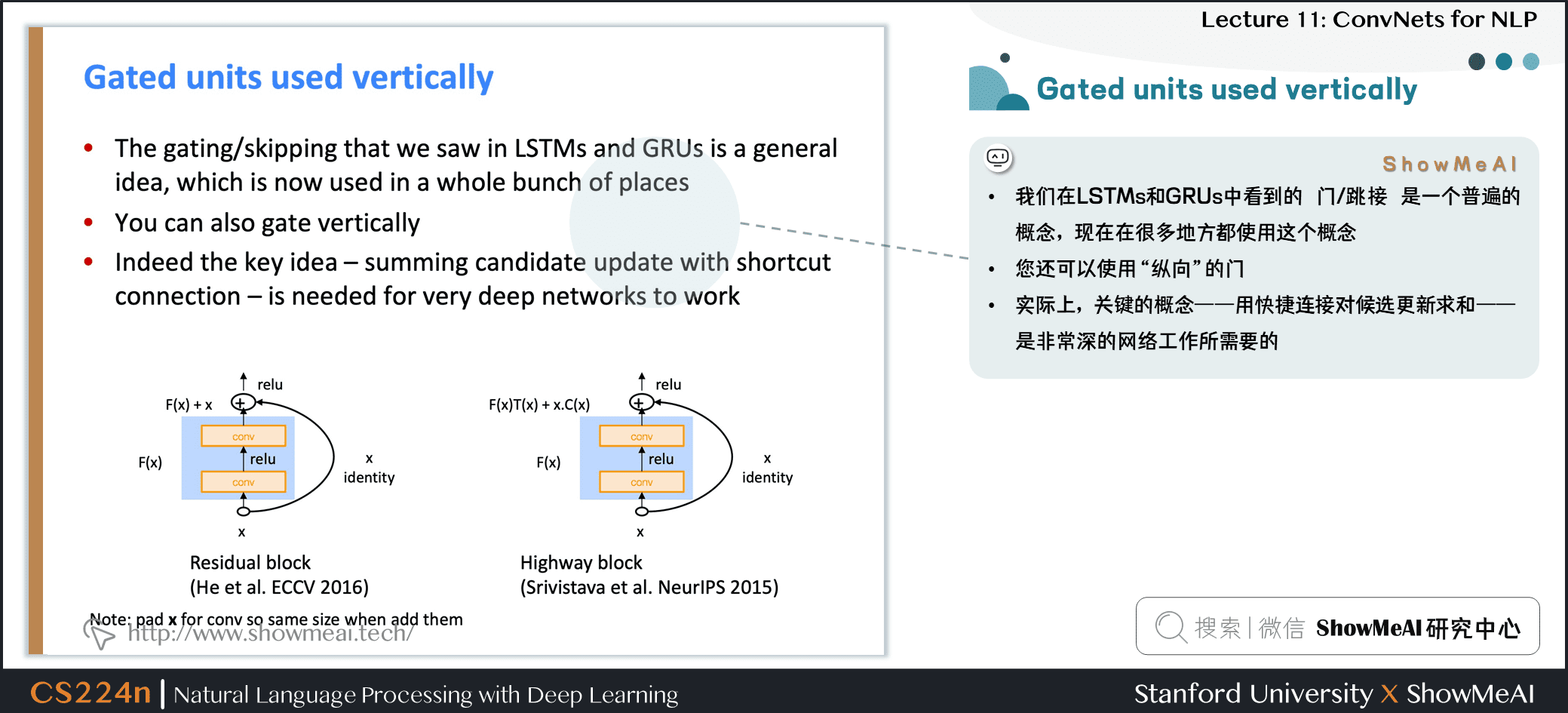• 我们在 LSTMs 和 GRUs 中看到的 门/跳接 是一个普遍的概念，现在在很多地方都使用这个概念

• 你还可以使用 **纵向** 的门

• 实际上，关键的概念——用快捷连接对候选更新求和——是非常深的网络工作所需要的

• Note：添加它们时，请将 xxx 填充成conv一样的维度，再求和

## 3.6 批归一化BatchNorm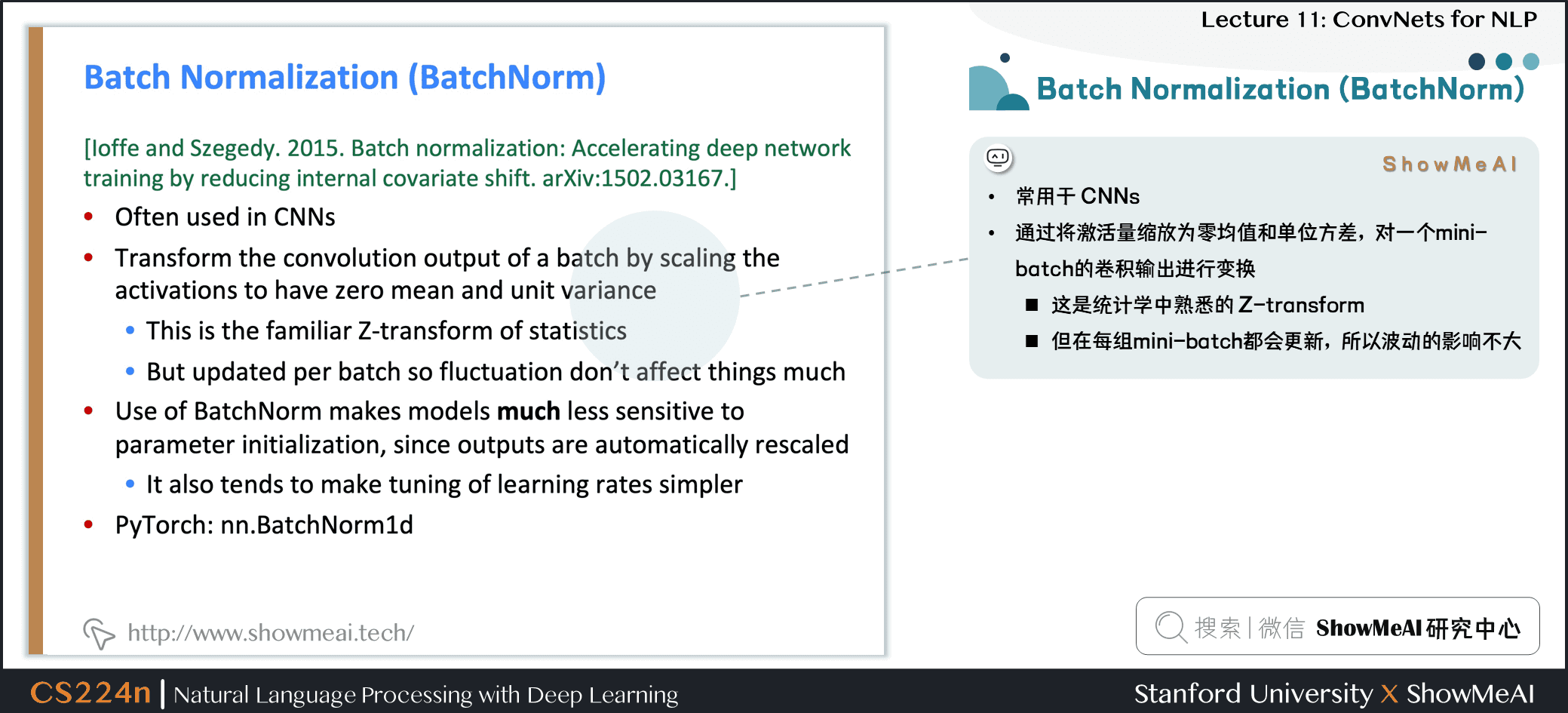• 常用于 CNNs

• 通过将激活量缩放为零均值和单位方差，对一个 mini-batch 的卷积输出进行变换

• 这是统计学中熟悉的 Z-transform
• 但在每组 mini-batch 都会更新，所以波动的影响不大
• 使用 BatchNorm 使模型对参数初始化的敏感程度下降，因为输出是自动重新标度的

• 也会让学习率的调优更简单，模型的训练会更加稳定
• PyTorch：nn.BatchNorm1d

## 3.7 1x1卷积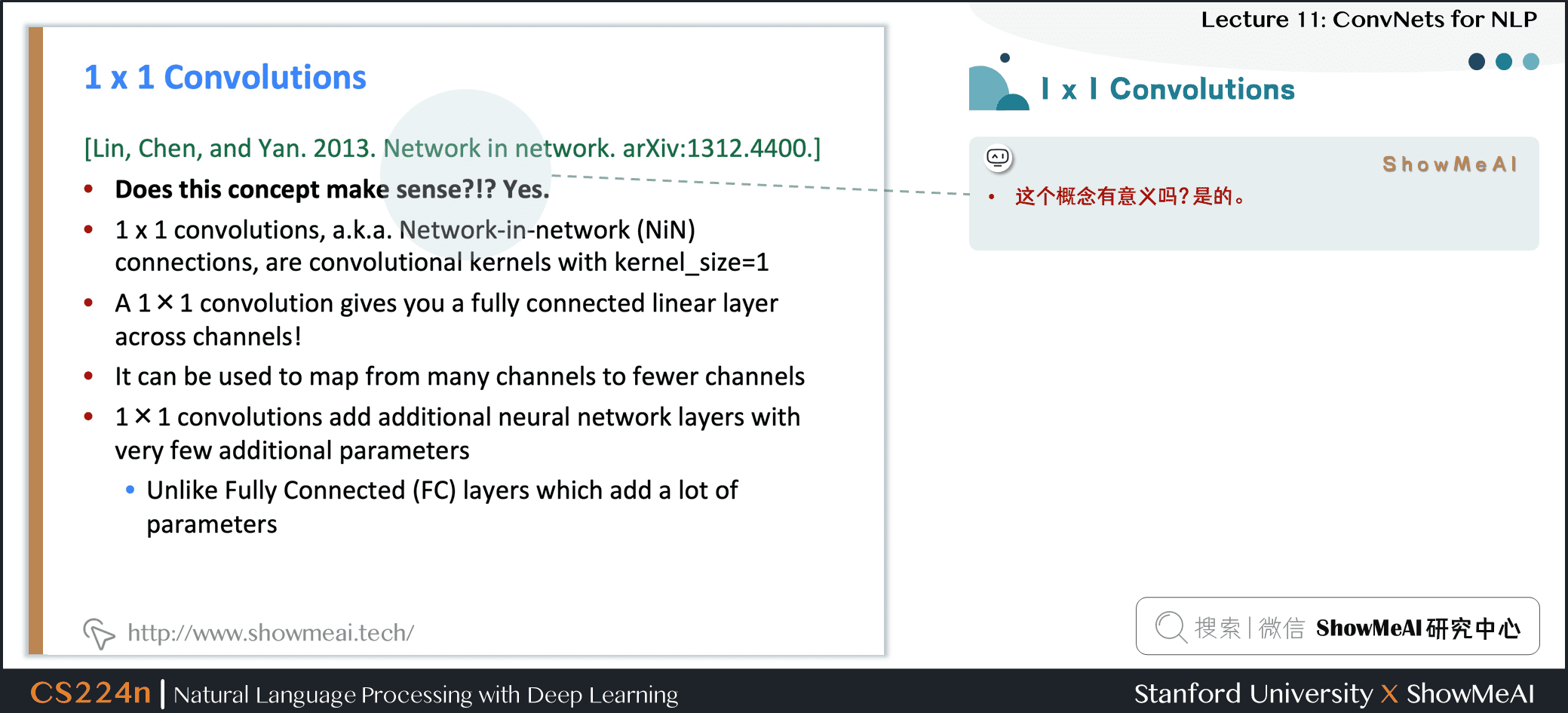• 1x1的卷积有作用吗是的

• 1x1 卷积，即网络中的 Network-in-network (NiN) connections，是内核大小为1的卷积内核

• 1x1 卷积提供了一个跨通道的全连接的线性层

• 它可以用于从多个通道映射到更少的通道

• 1x1 卷积添加了额外的神经网络层，附加的参数很少

• 与全连接 (FC) 层不同——全连接(FC)层添加了大量的参数

## 3.8 CNN 应用：机器翻译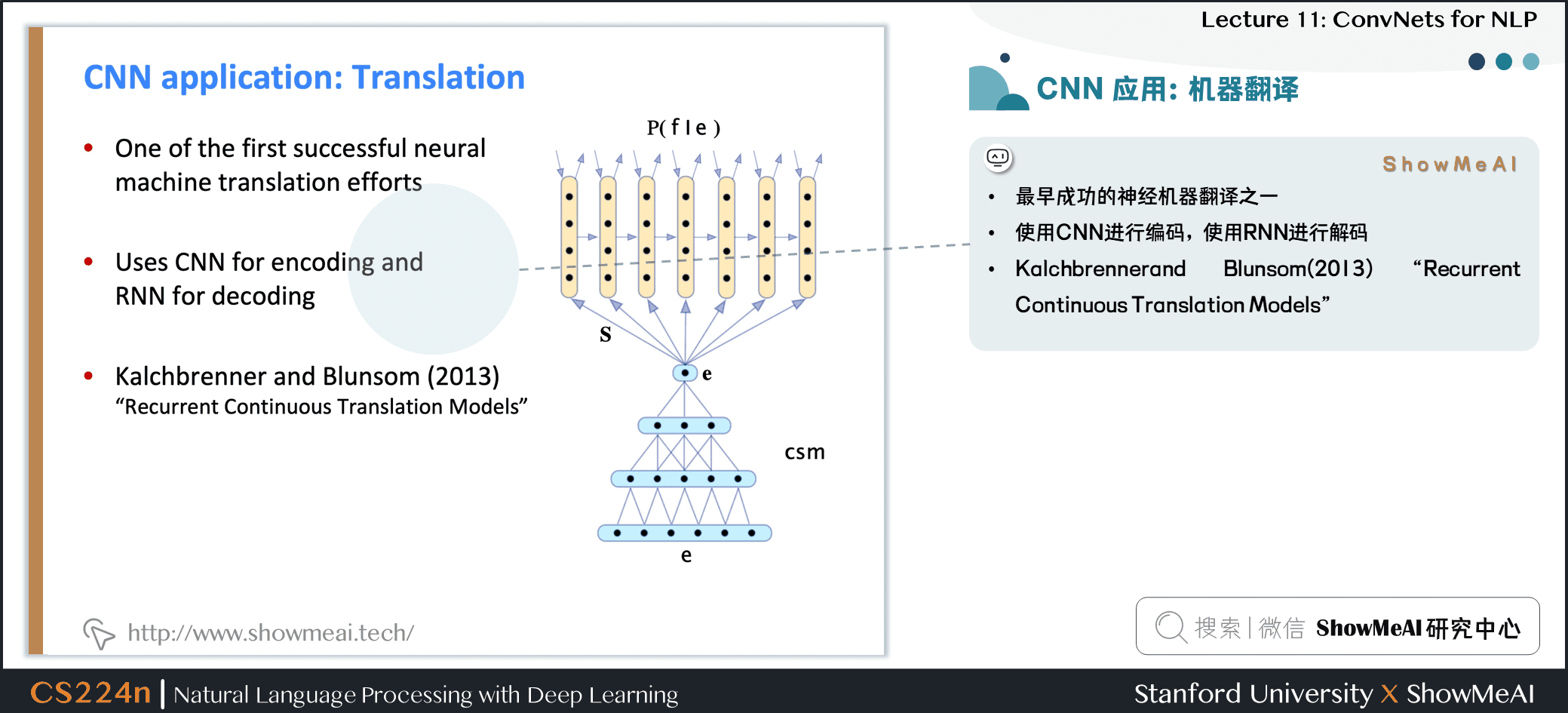• 最早成功的神经机器翻译之一
• 使用CNN进行编码，使用RNN进行解码
• Kalchbrennerand Blunsom(2013) Recurrent Continuous Translation Models

## 3.9 #论文解读# Learning Character-level Representations for Part-of-Speech Tagging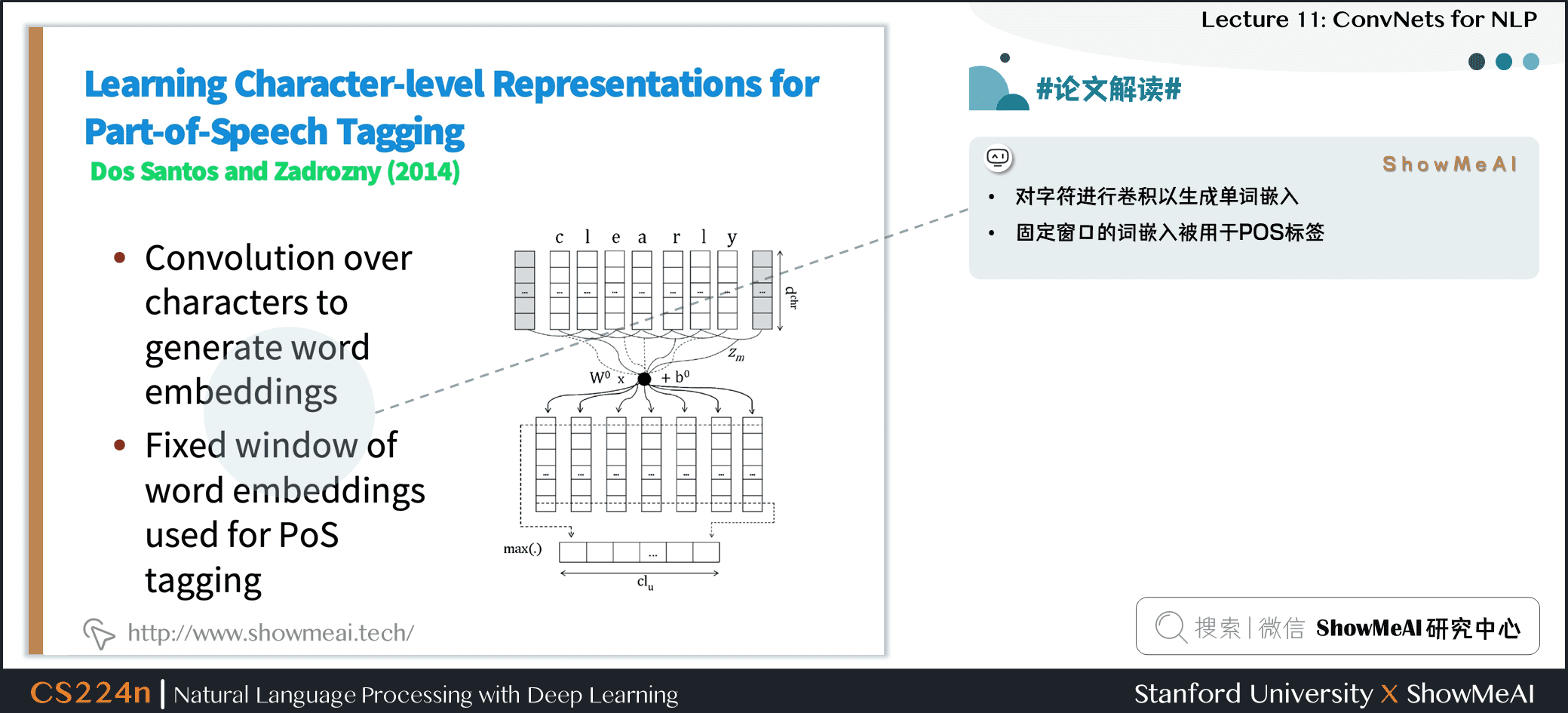• 对字符进行卷积以生成单词嵌入
• 固定窗口的词嵌入被用于 POS 标签

## 3.10 #论文解读# Character-Aware Neural Language Models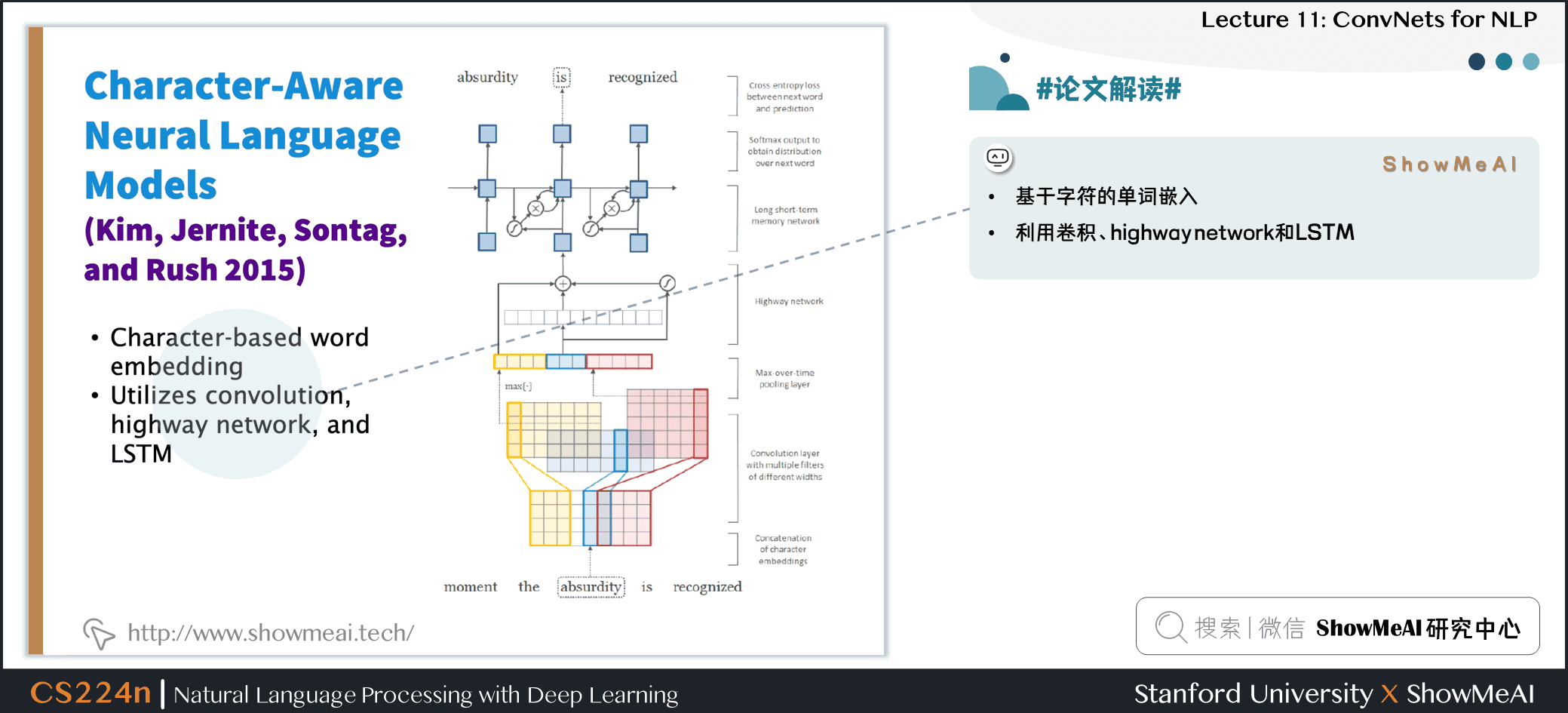• 基于字符的单词嵌入
• 利用卷积、highway network 和 LSTM

# 4.深度CNN用于文本分类

## 4.1 深度卷积网络用于文本分类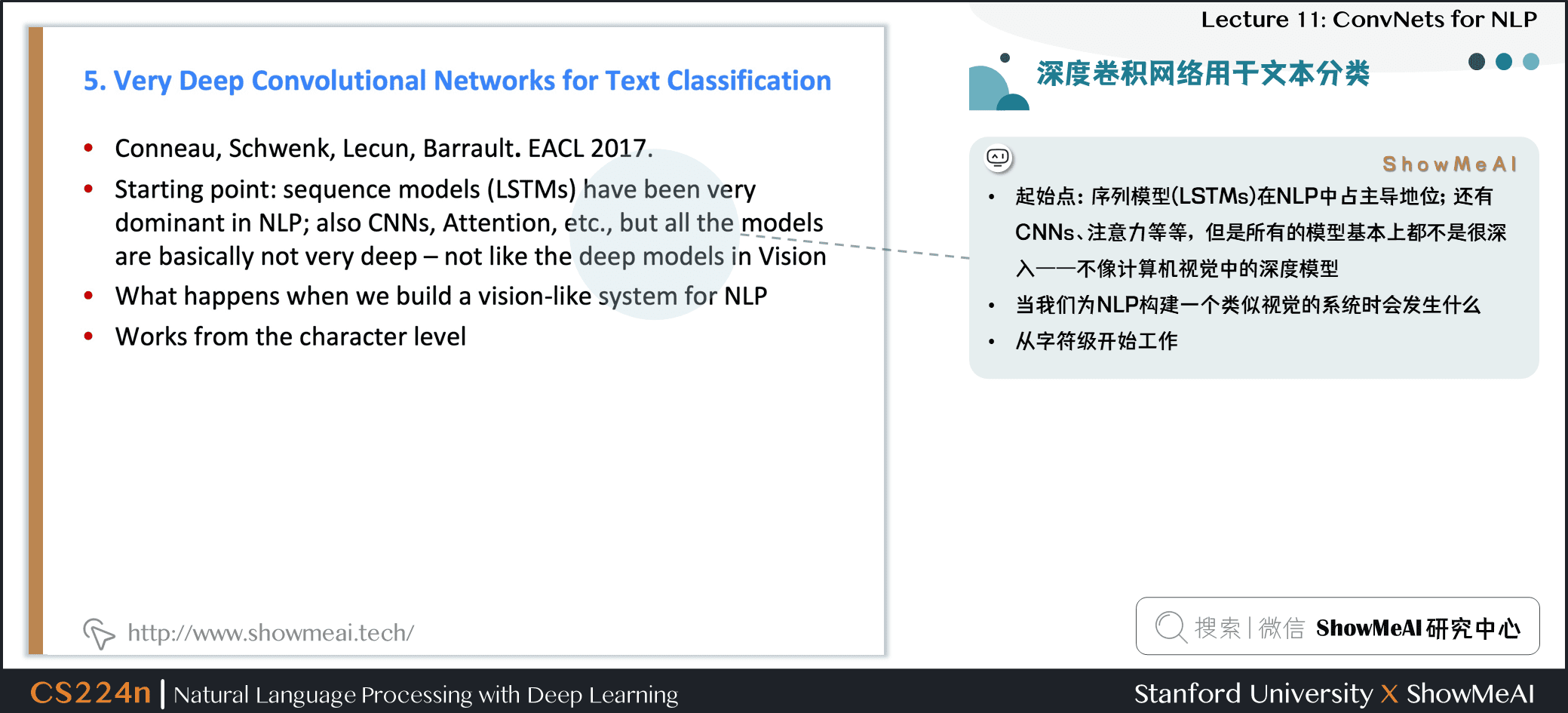• 起始点：序列模型 (LSTMs) 在 NLP 中占主导地位；还有CNNs、注意力等等，但是所有的模型基本上都不是很深入——不像计算机视觉中的深度模型
• 当我们为 NLP 构建一个类似视觉的系统时会发生什么
• 从字符级开始工作

## 4.2 VD-CNN 结构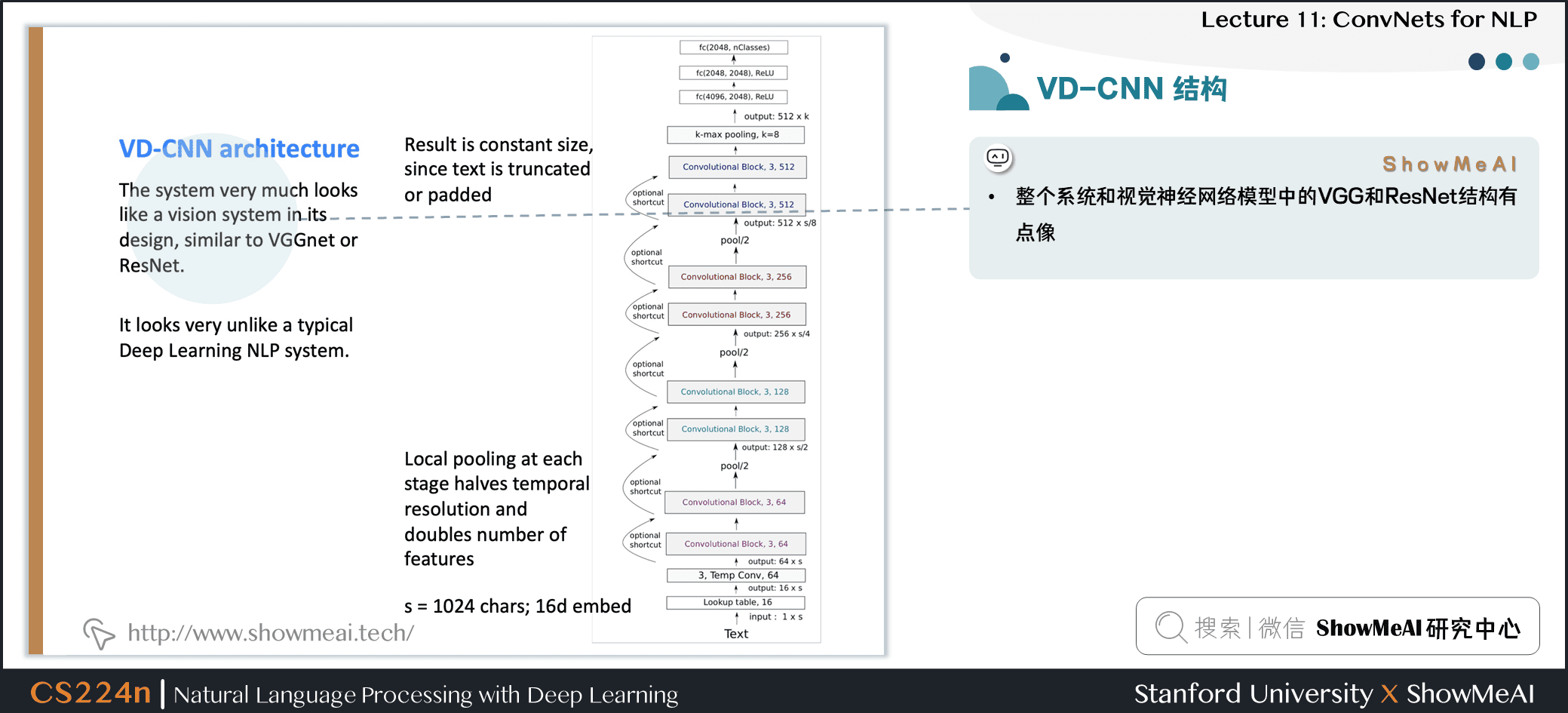• 整个系统和视觉神经网络模型中的 VGG 和 ResNet 结构有点像

• 不太像一个典型的深度学习 NLP 系统

• 结果是固定大小，因为文本被截断或者填充成统一长度了

• 每个阶段都有局部池化操作，特征数量 double

## 4.3 VD-CNN的卷积模块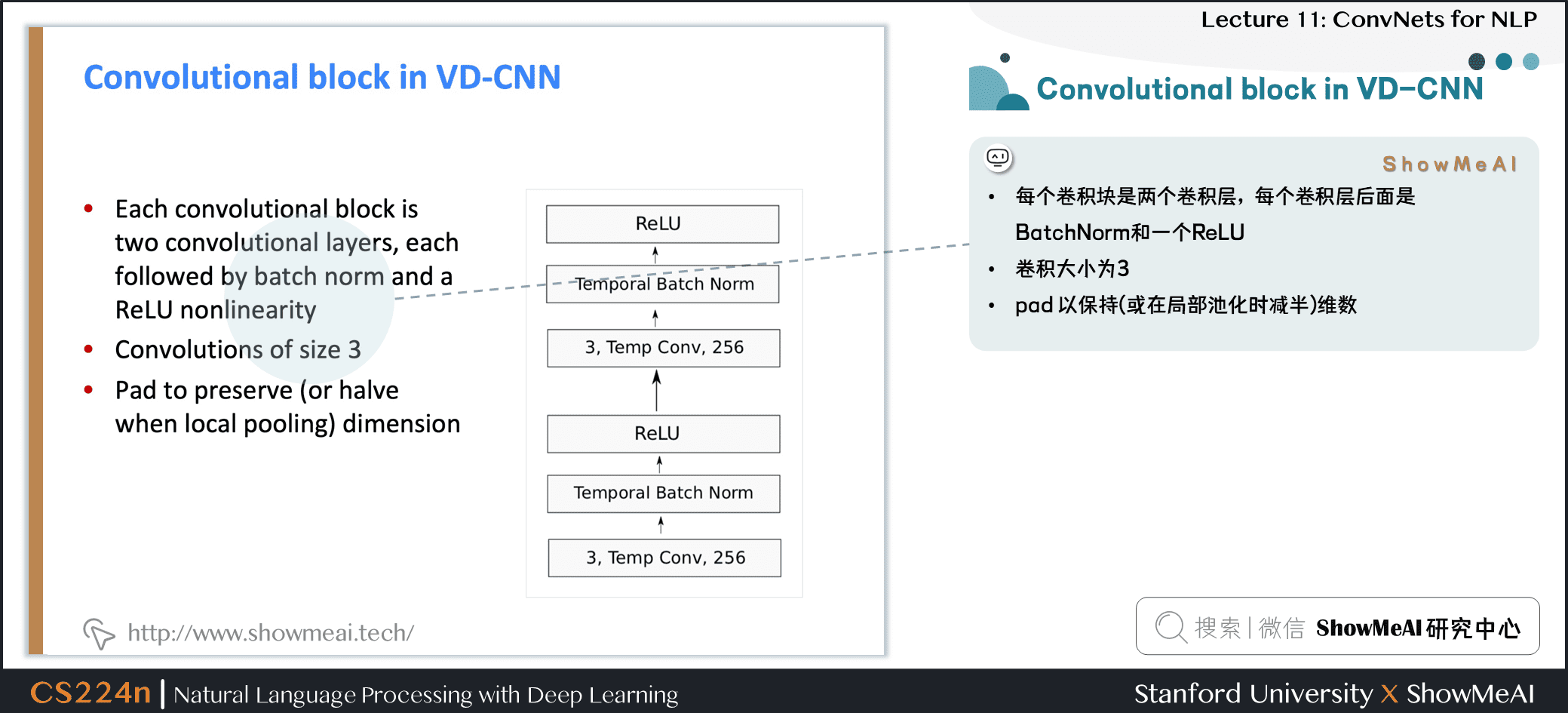• 每个卷积块是两个卷积层，每个卷积层后面是 BatchNorm 和一个 ReLU
• 卷积大小为 3

## 4.4 实验结果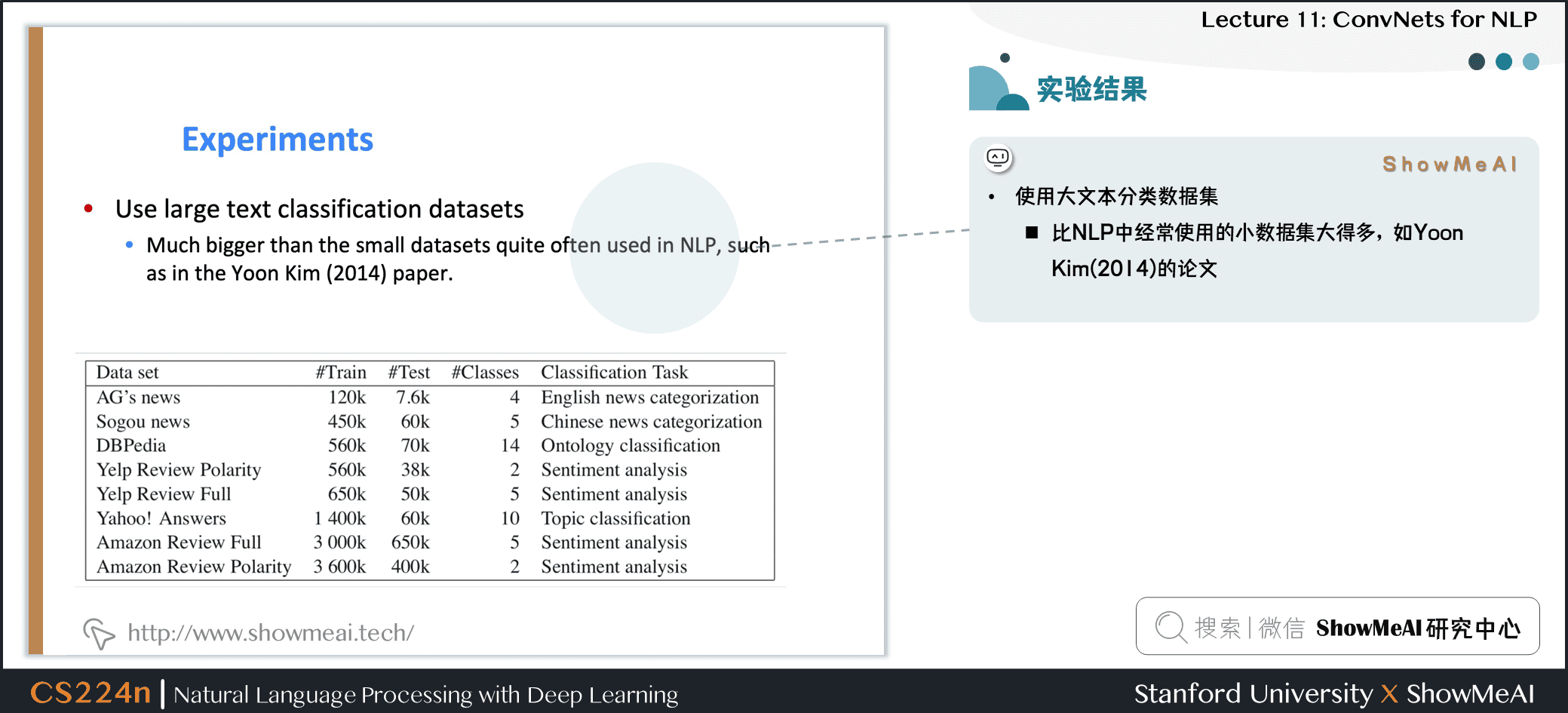• 使用大文本分类数据集
• 比 NLP 中经常使用的小数据集大得多，如Yoon Kim(2014) 的论文

• 以上数据均为错误率，所以越低越好
• 深度网络会取得更好的结果，残差层取得很好的结果，但是深度再深时并未取得效果提升
• 实验表明使用 MaxPooling 比 KMaxPooling 和 使用 stride 的卷积 的两种其他池化方法要更好
• ConvNets 可以帮助我们建立很好的文本分类系统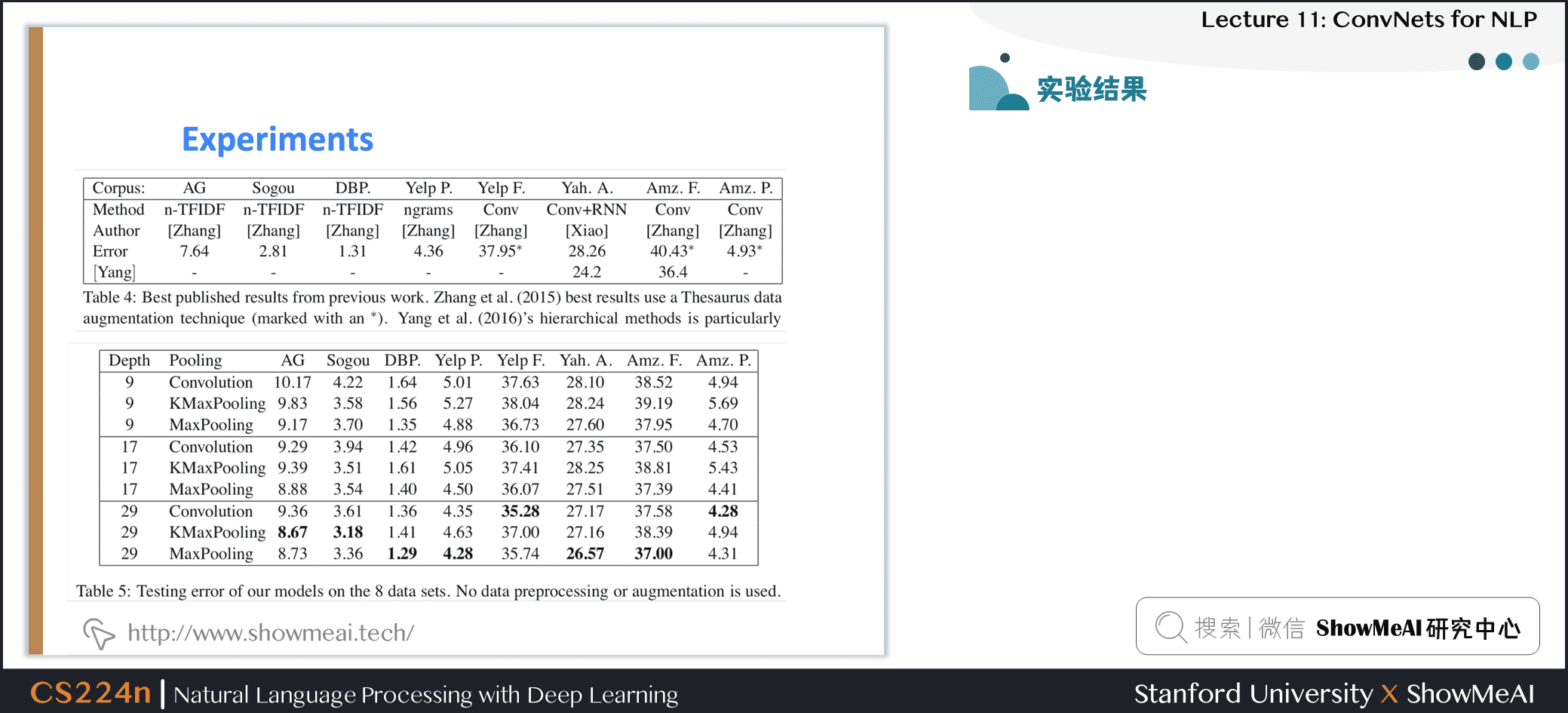## 4.5 RNNs比较慢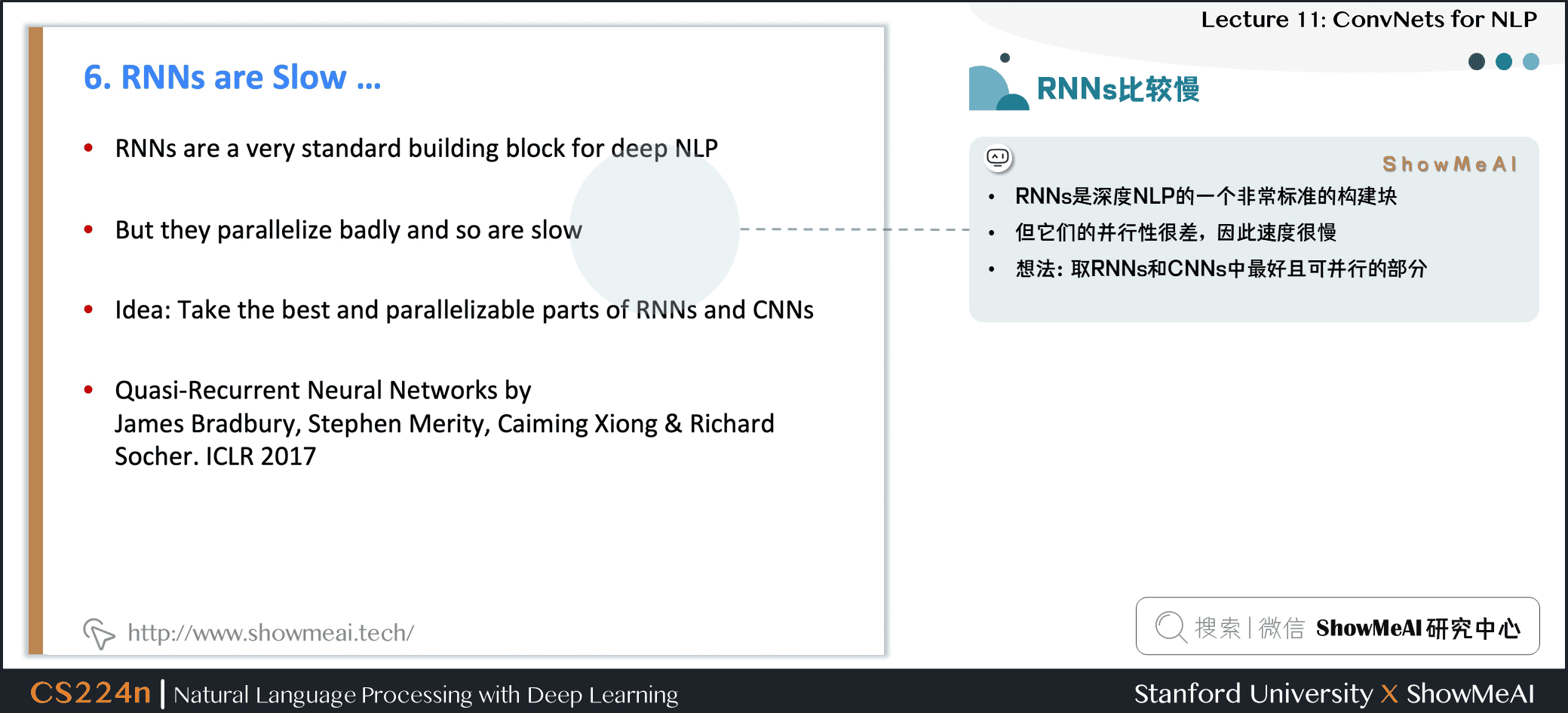• RNNs 是深度 NLP 的一个非常标准的构建块
• 但它们的并行性很差，因此速度很慢
• 想法：取 RNNs 和 CNNs 中最好且可并行的部分

# 5.Q-RNN模型

## 5.1 Quasi-Recurrent Neural Network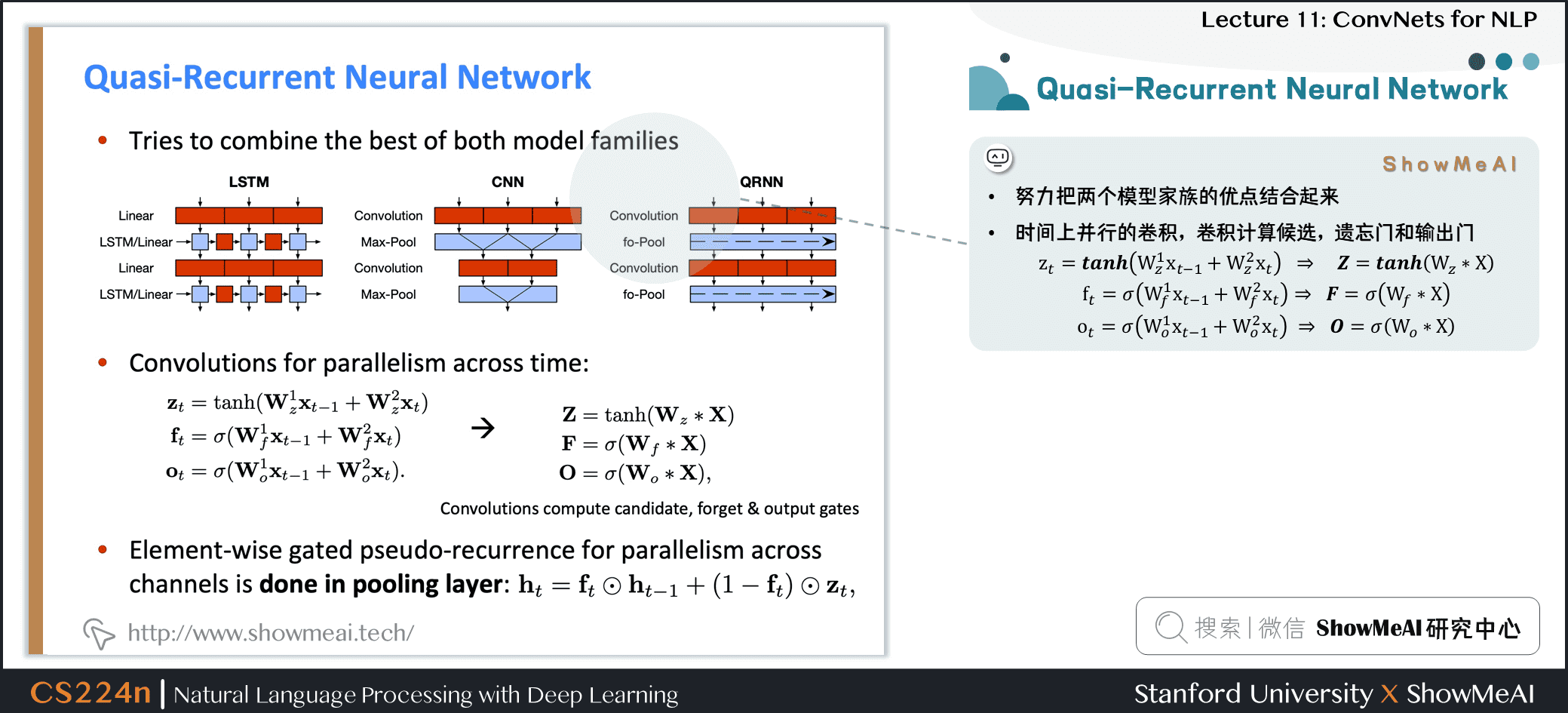• 努力把两个模型家族的优点结合起来
• 时间上并行的卷积，卷积计算候选，遗忘门和输出门

ztftot=tanh(W1zxt−1+W2zxt)=σ(W1fxt−1+W2fxt)=σ(W1oxt−1+W2oxt)zt=tanh⁡(Wz1xt−1+Wz2xt)ft=σ(Wf1xt−1+Wf2xt)ot=σ(Wo1xt−1+Wo2xt)\begin{aligned}
\mathbf{z}_{t} &=\tanh \left(\mathbf{W}_{z}^{1} \mathbf{x}_{t-1}+\mathbf{W}_{z}^{2} \mathbf{x}_{t}\right) \
\mathbf{f}_{t} &=\sigma\left(\mathbf{W}_{f}^{1} \mathbf{x}_{t-1}+\mathbf{W}_{f}^{2} \mathbf{x}_{t}\right) \
\mathbf{o}_{t} &=\sigma\left(\mathbf{W}_{o}^{1} \mathbf{x}_{t-1}+\mathbf{W}_{o}^{2} \mathbf{x}_{t}\right)
\end{aligned}
ZFO=tanh(Wz∗X)=σ(Wf∗X)=σ(Wo∗X)Z=tanh⁡(Wz∗X)F=σ(Wf∗X)O=σ(Wo∗X)\begin{aligned}
\mathbf{Z} &=\tanh \left(\mathbf{W}_{z} \mathbf{X}\right) \
\mathbf{F} &=\sigma\left(\mathbf{W}_{f}
\mathbf{X}\right) \
\mathbf{O} &=\sigma\left(\mathbf{W}_{o} * \mathbf{X}\right)
\end{aligned}

• 跨通道并行性的逐元素的门控伪递归是在池化层中完成的

ht=ft⊙ht−1+(1−ft)⊙ztht=ft⊙ht−1+(1−ft)⊙zt\mathbf{h}_{t}=\mathbf{f}_{t} \odot \mathbf{h}_{t-1}+\left(1-\mathbf{f}_{t}\right) \odot \mathbf{z}_{t}

## 5.2 Q-RNN 实验：语言模型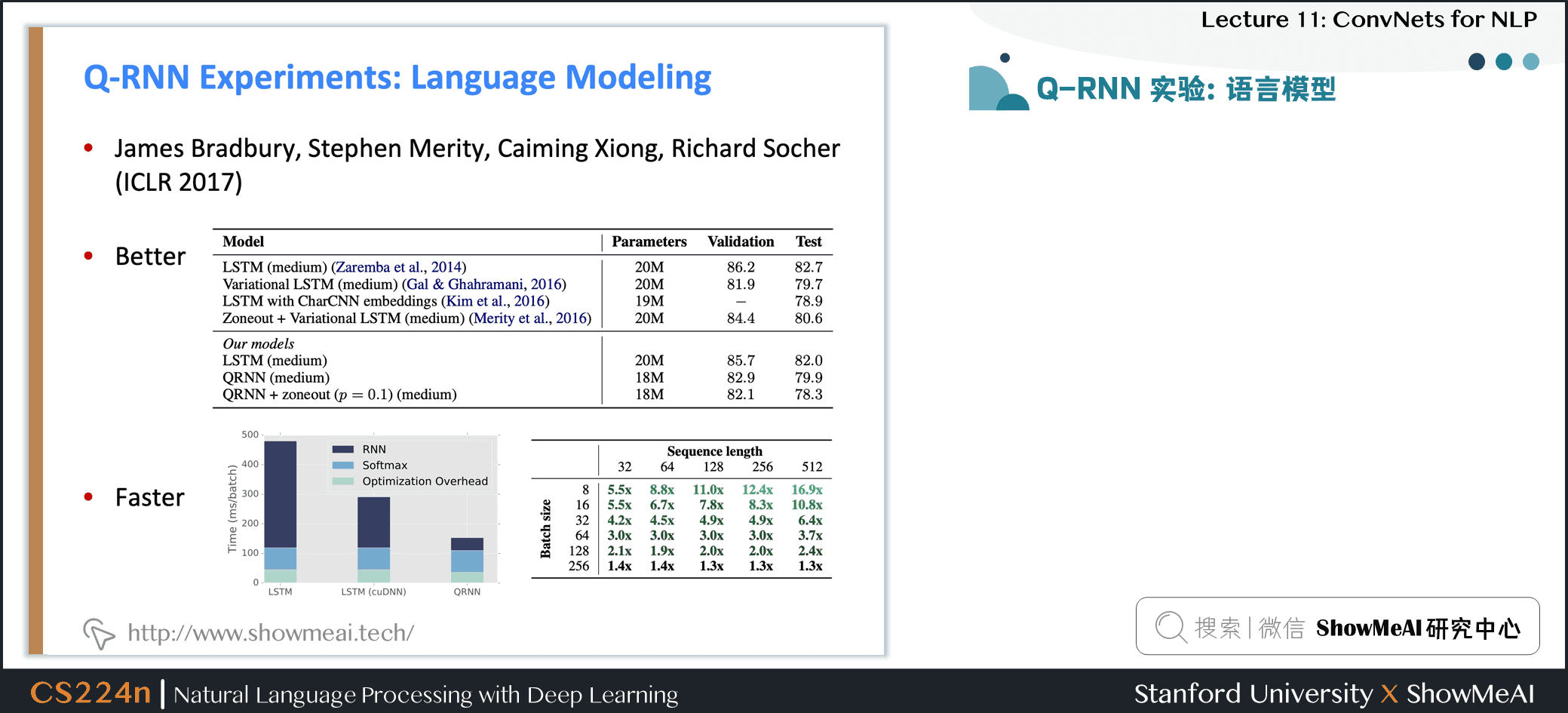## 5.3 Q-RNNs：情感分析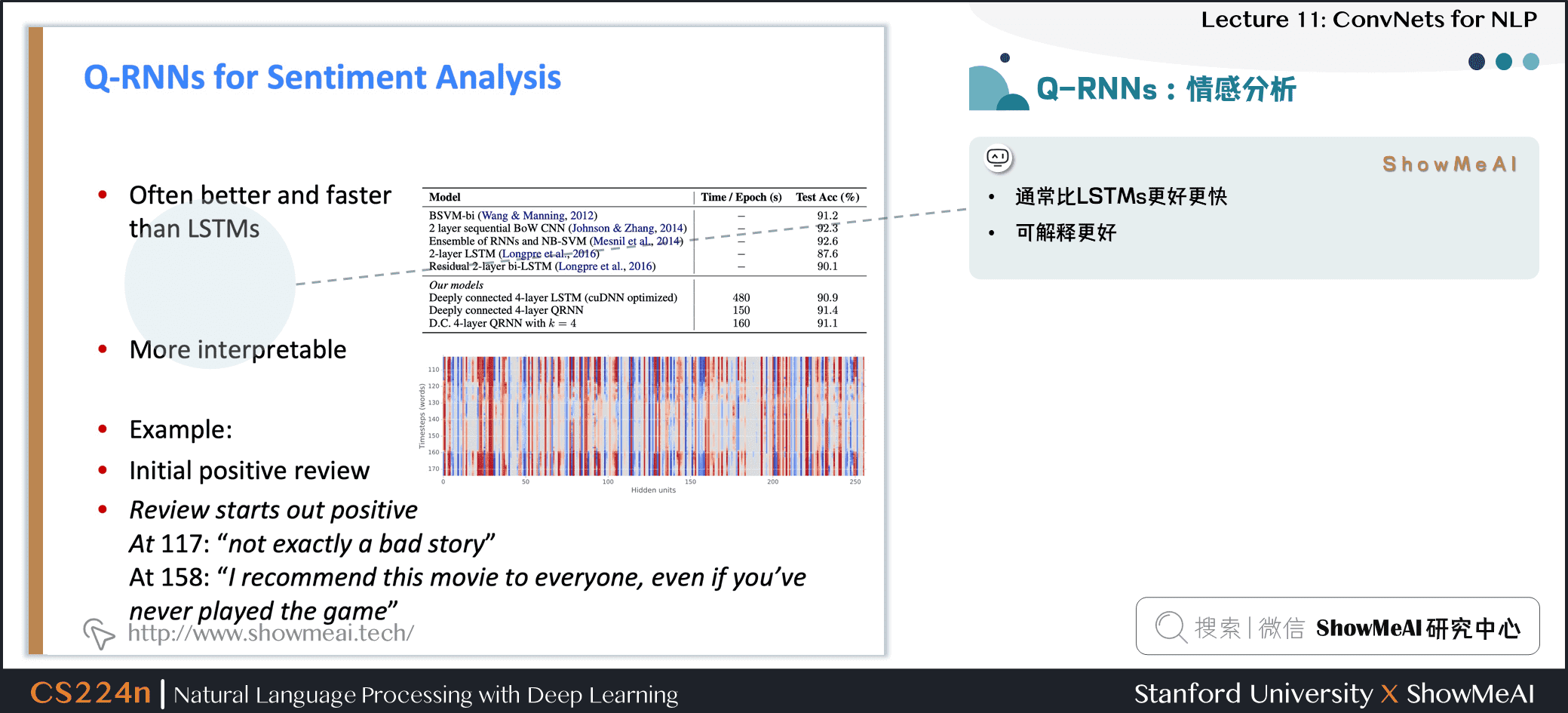• 通常比 LSTMs 更好更快
• 可解释更好

## 5.4 QRNN 的限制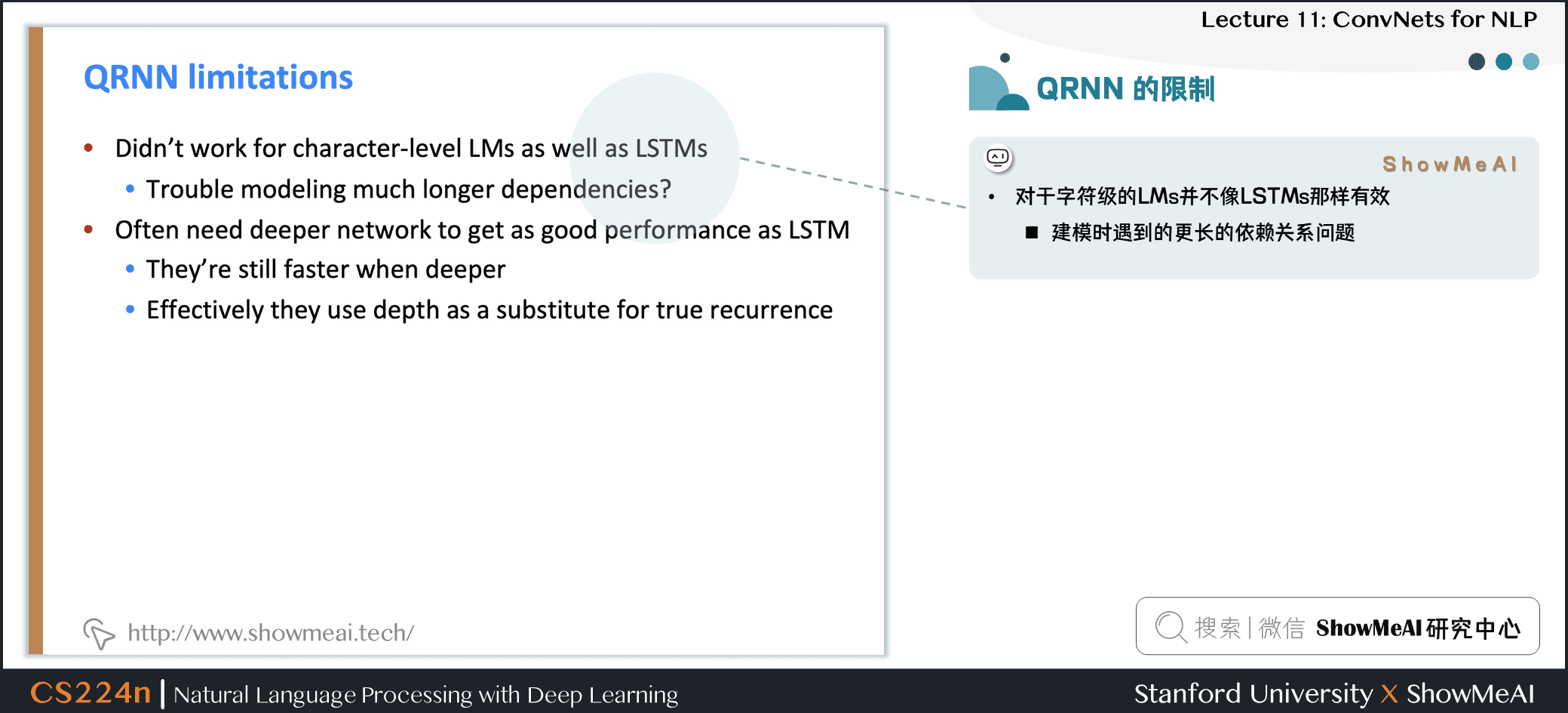• 对于字符级的 LMs 并不像 LSTMs 那样有效

• 建模时遇到的更长的依赖关系问题
• 通常需要更深入的网络来获得与 LSTM 一样好的性能

• 当它们更深入时，速度仍然更快
• 有效地使用深度作为真正递归的替代

## 5.5 RNN的缺点&Transformer提出的动机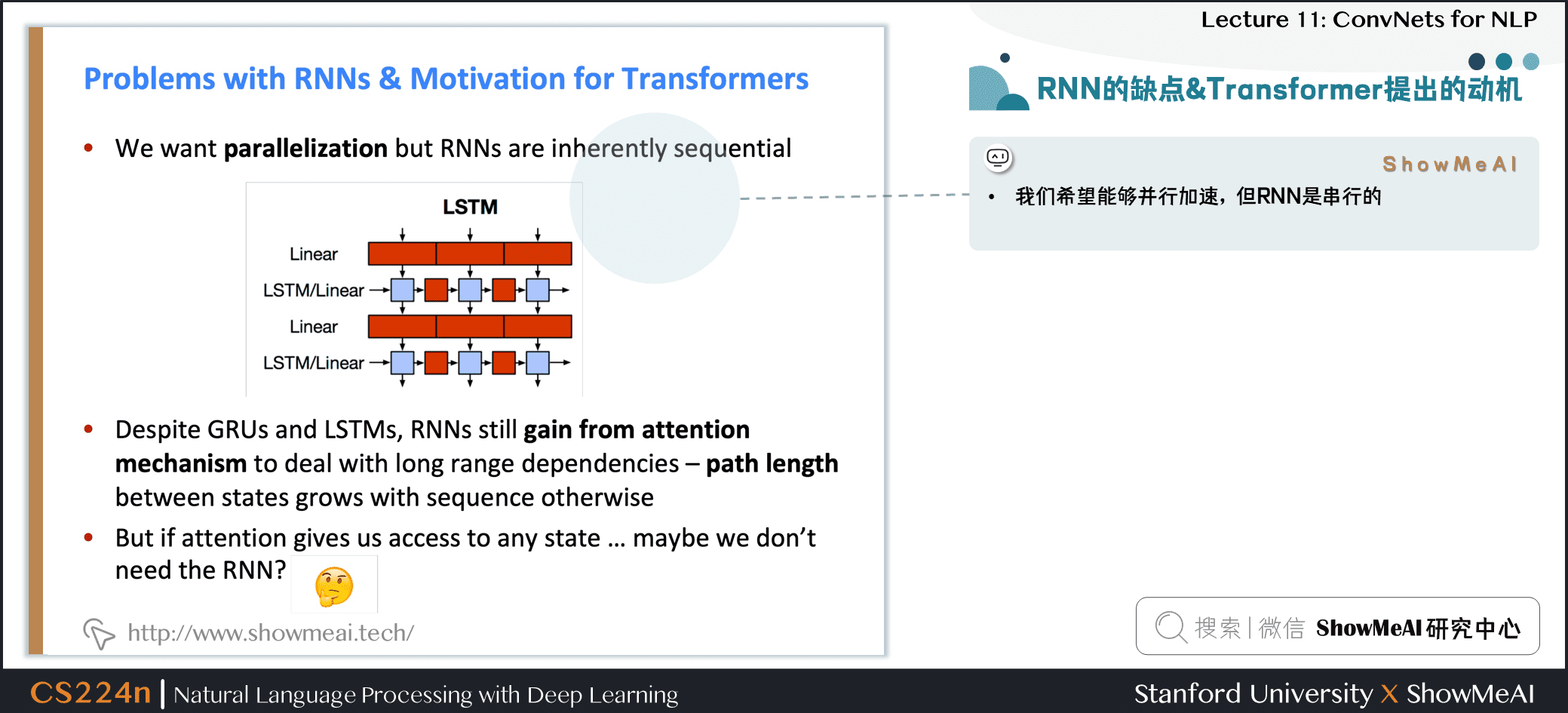• 我们希望能够并行加速，但 RNN 是串行的

• 尽管 GRUs 和 LSTMs，RNNs 通过注意力机制可以捕捉到长时依赖，但随着序列增长，需要计算的路径也在增长

• 如果注意力机制本身可以让我们关注任何位置的信息，可能我们不需要 RNN？

# 6.视频教程

[video(video-klkA71cI-1652090215444)(type-bilibili)(url-https://player.bilibili.com/player.html?aid=376755412&page=11)(image-https://img-blog.csdnimg.cn/img_convert/7ee2eb31a0061266f88507f561dd96f4.png)(title-【双语字幕+资料下载】斯坦福CS224n | 深度学习与自然语言处理(2019·全20讲))]

# 斯坦福 CS224n 课程带学详解